• 沃尔什哈达玛变换 MFC对话框程序，想要资源的拿去学习把
• 沃尔什哈达玛变换算法说明，来源于数字图像处理第七章ppt资源
• 余弦变换因为只有实变换，所以只有单个幅频谱，而且显然比傅里叶变换更加集中（并且在一个点，而不是四个点） JPG等都用的是余弦变换 为什么分成小块，因为大块大大增加运算量 MATLAB调用d...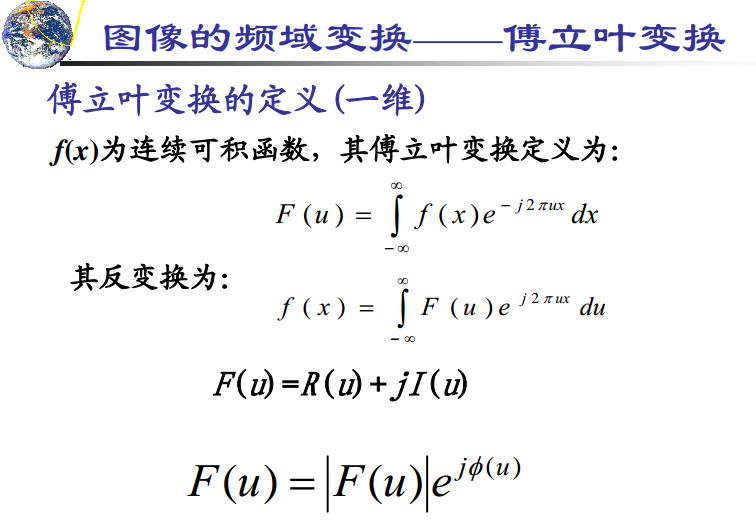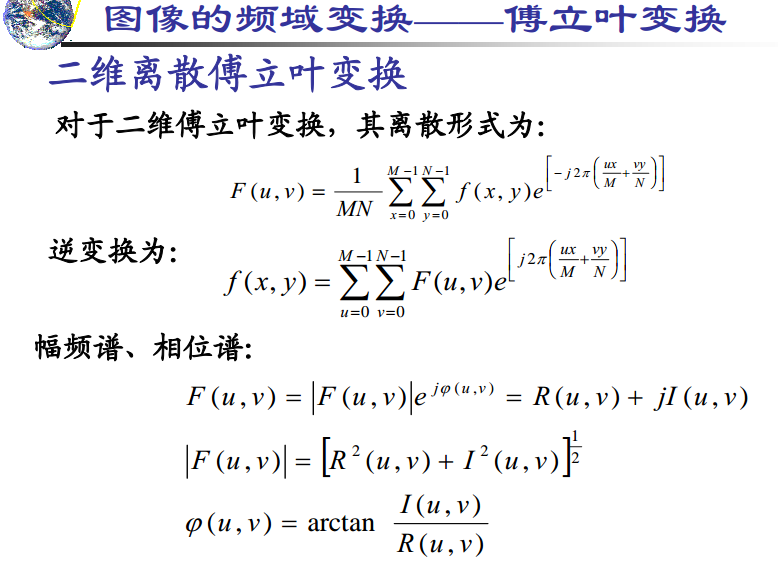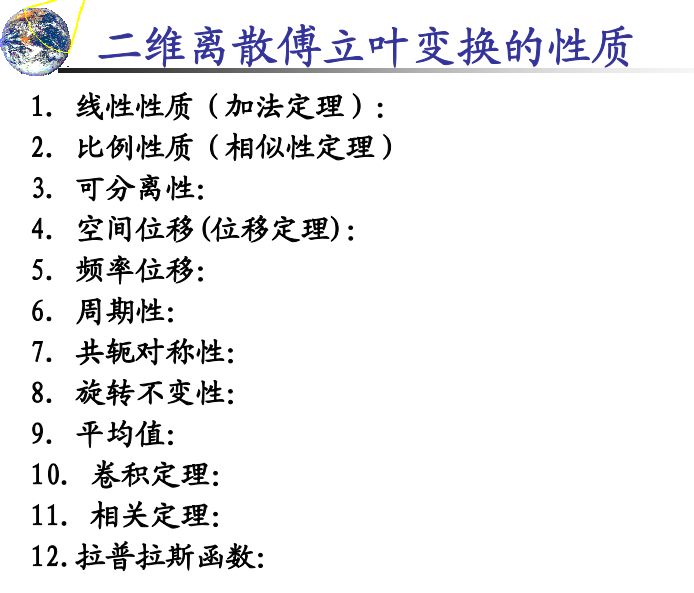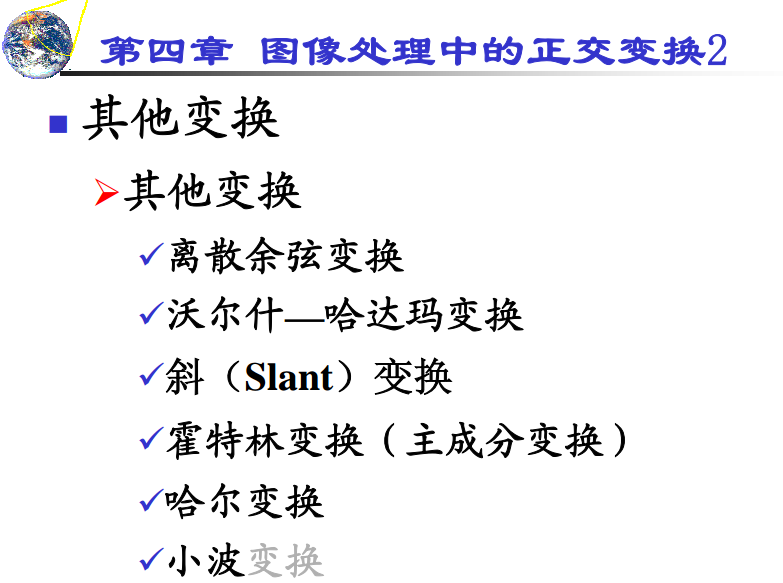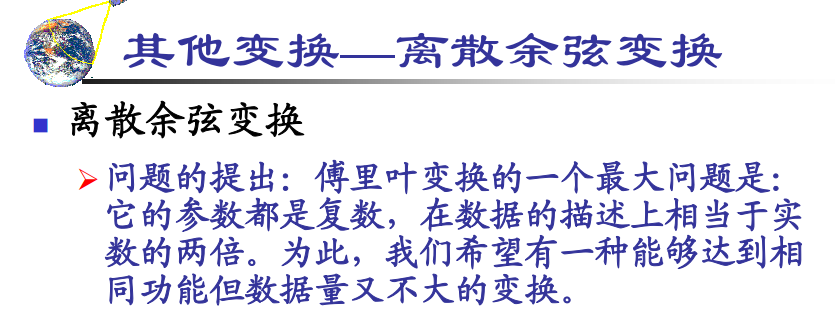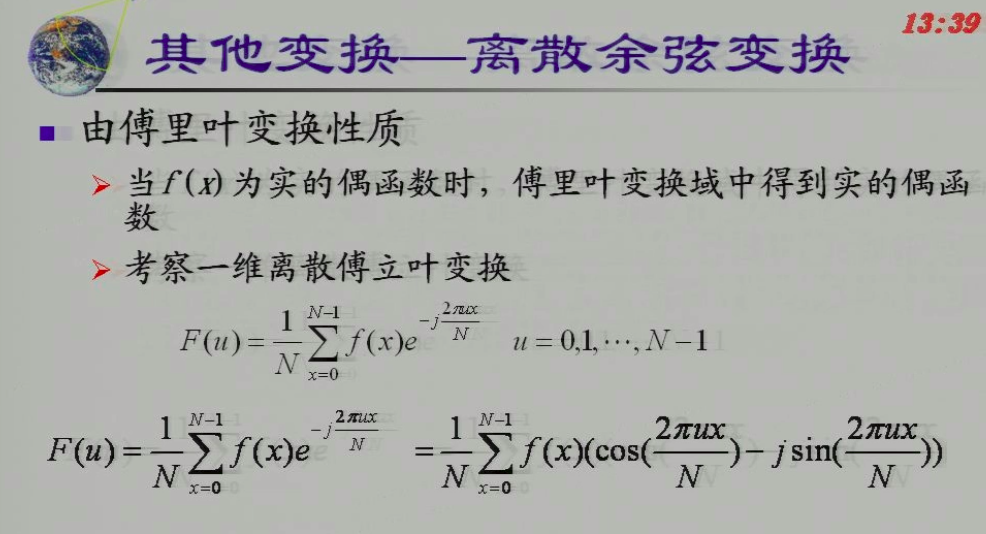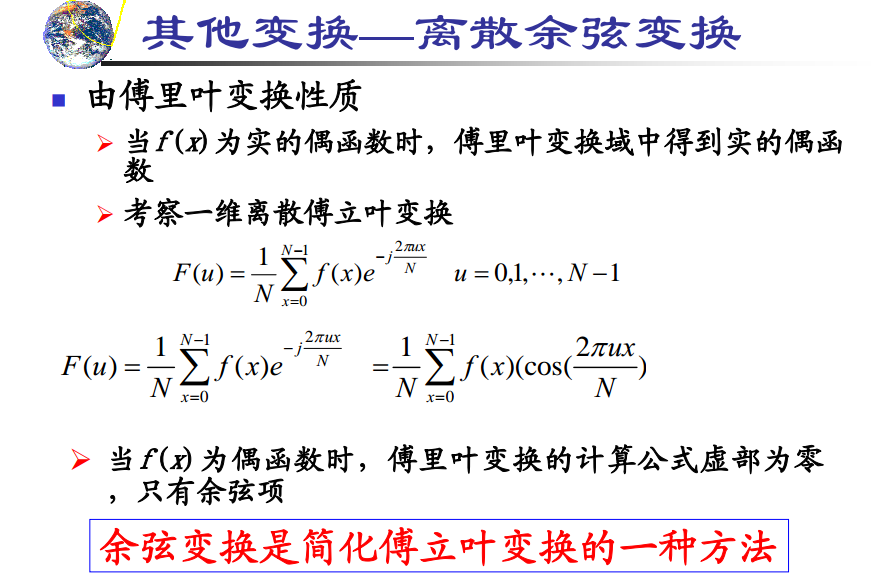实函数的要求已经达到，但是偶函数的要求比较难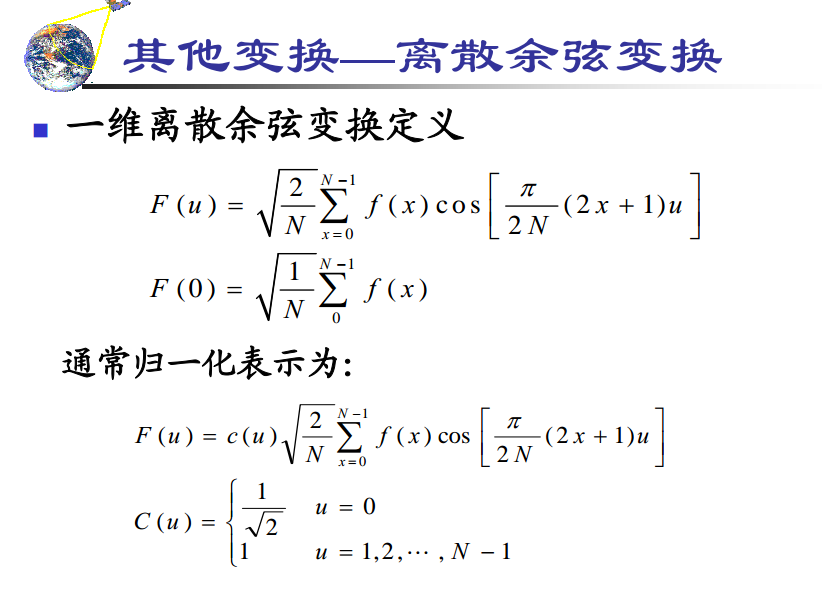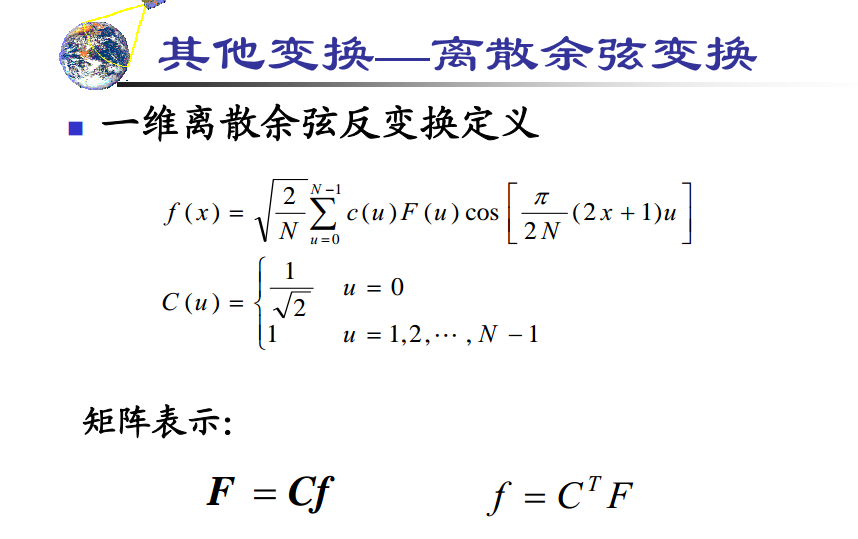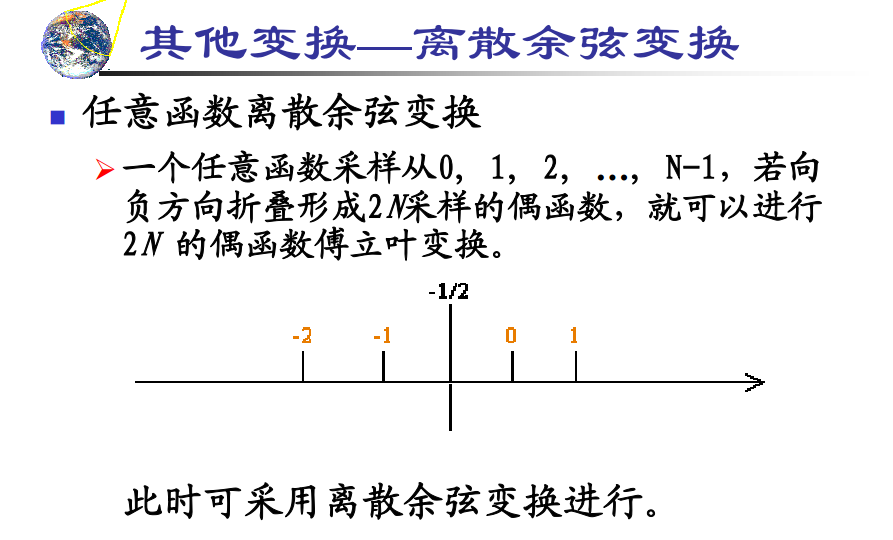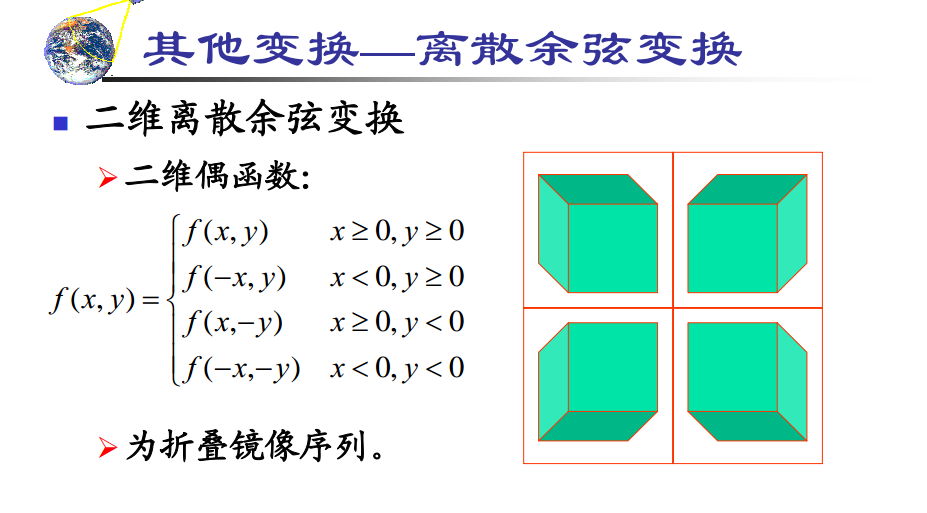假定具有这种对称性，真实情况比会有误差，但是误差可以忽略不计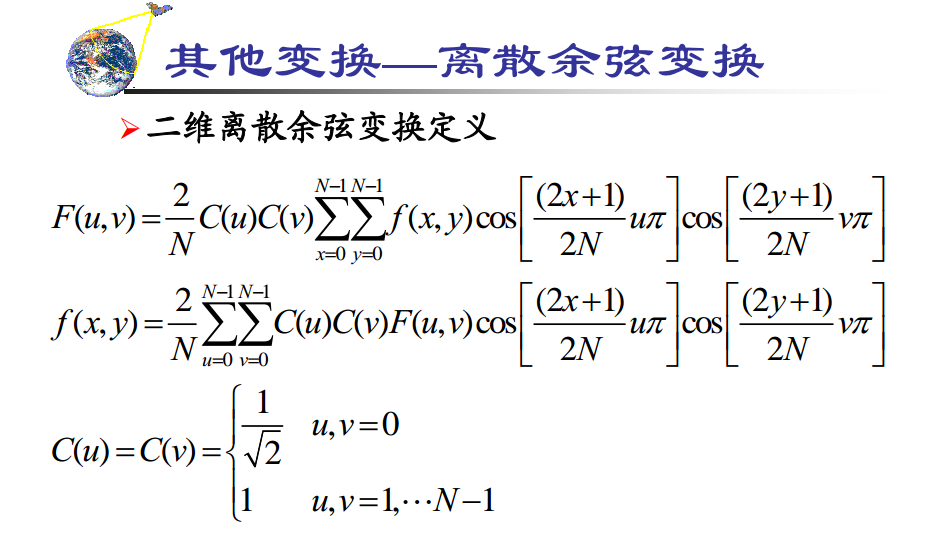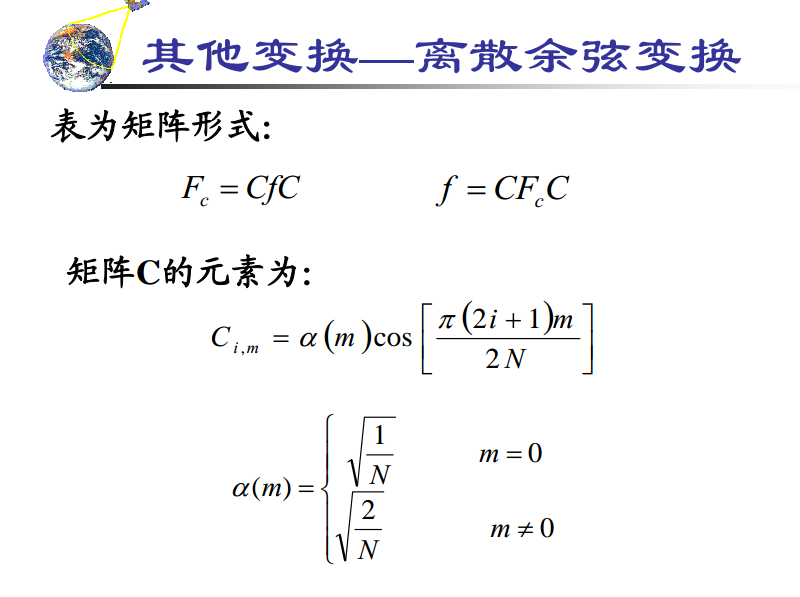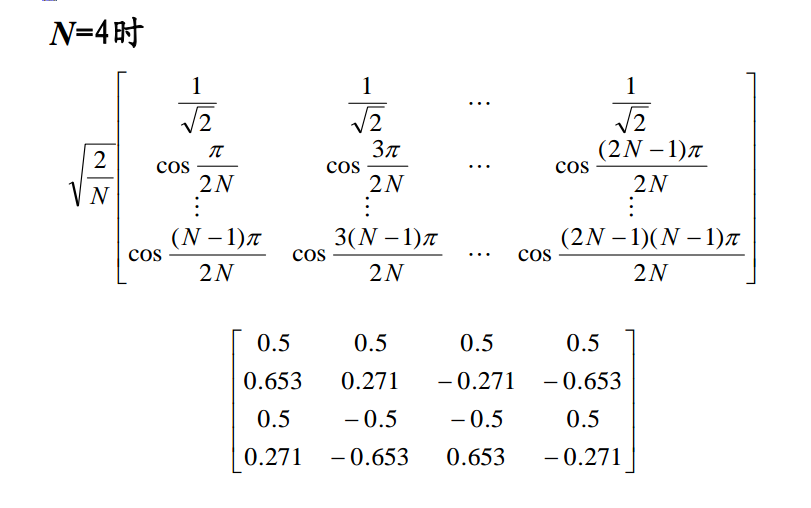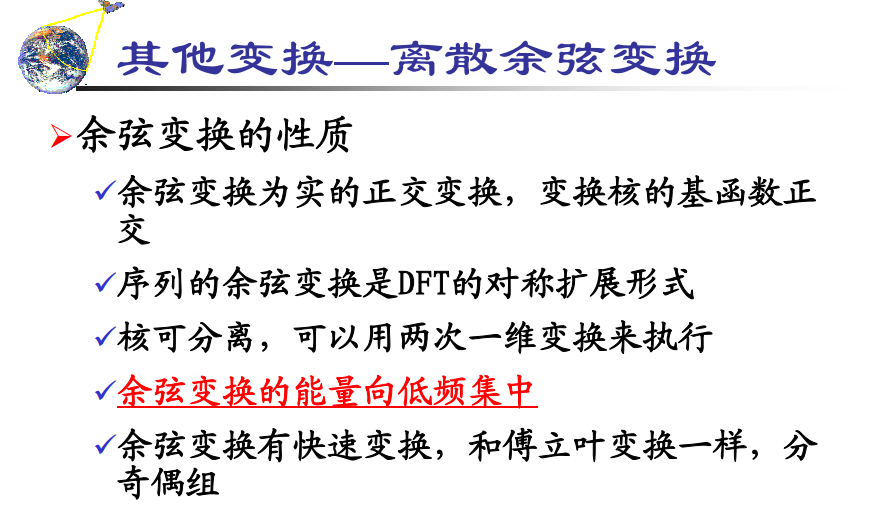余弦变换因为只有实变换，所以只有单个幅频谱，而且显然比傅里叶变换更加集中（并且在一个点，而不是四个点）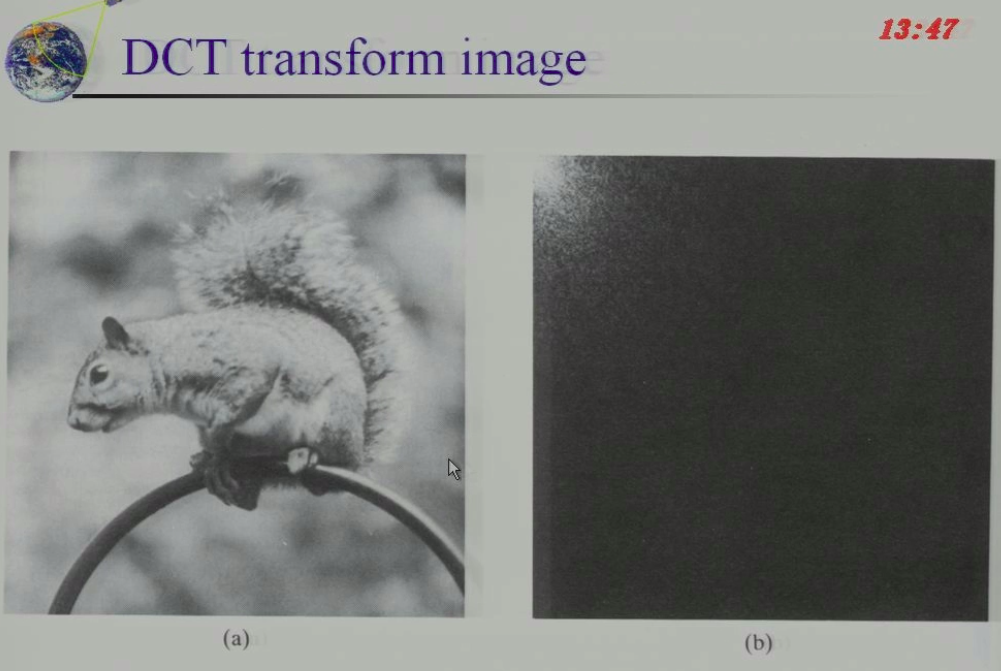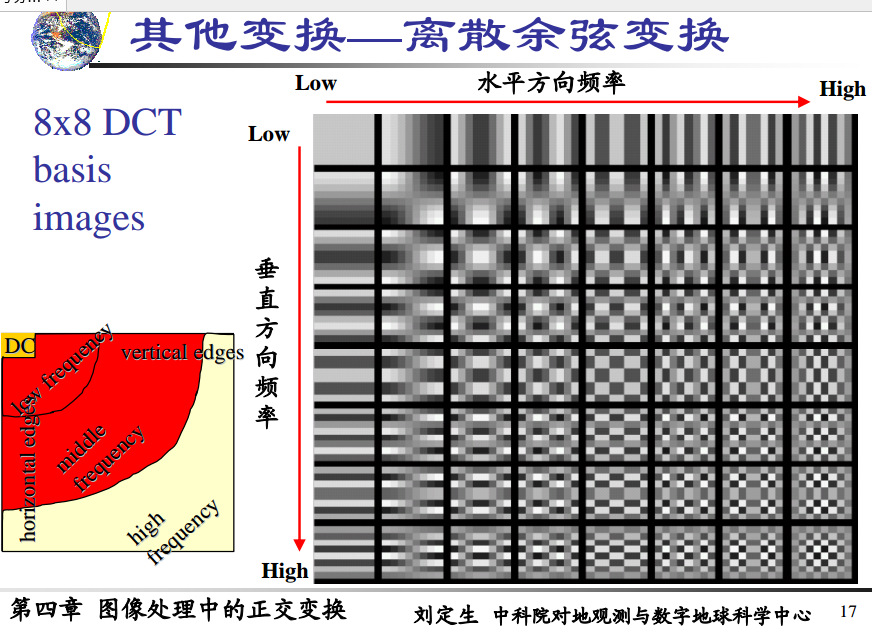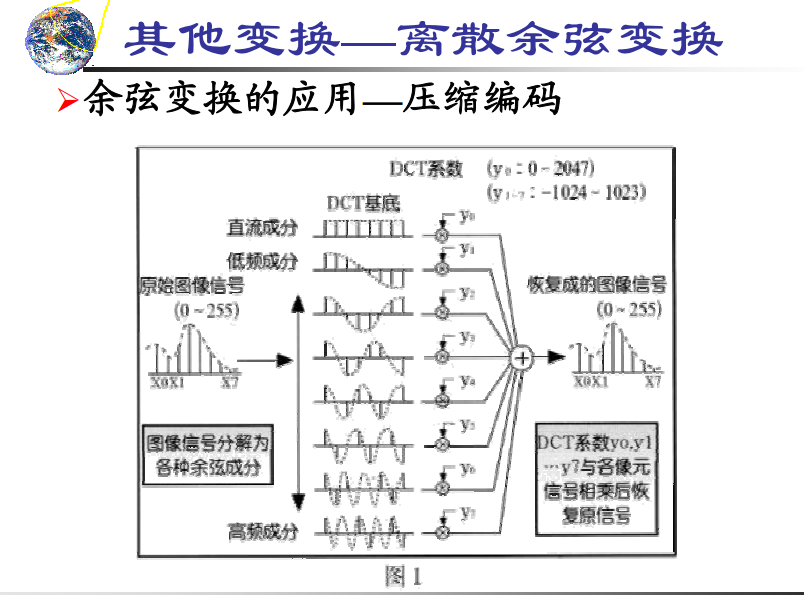JPG等都用的是余弦变换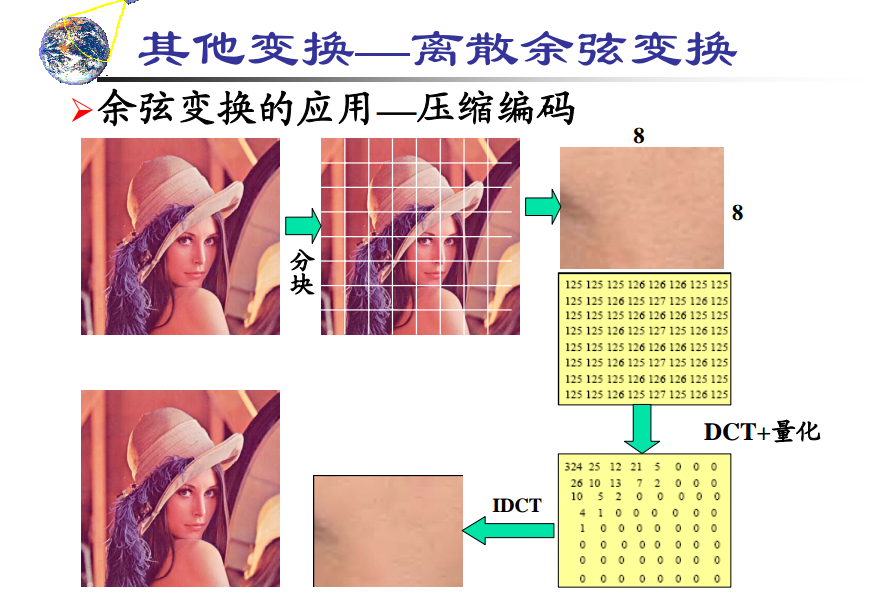为什么分成小块，因为大块大大增加运算量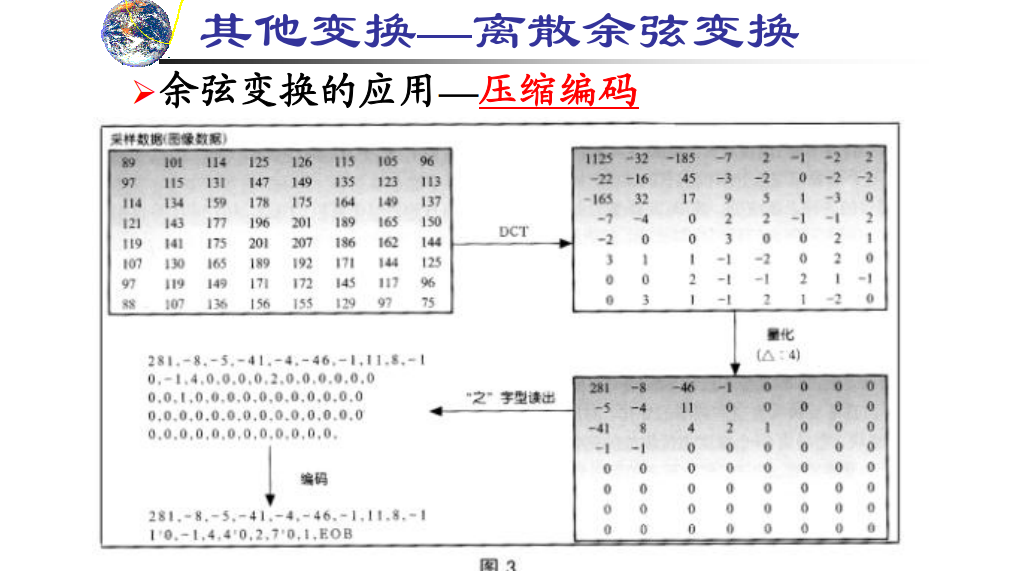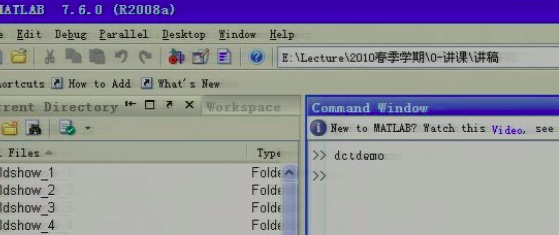MATLAB调用demo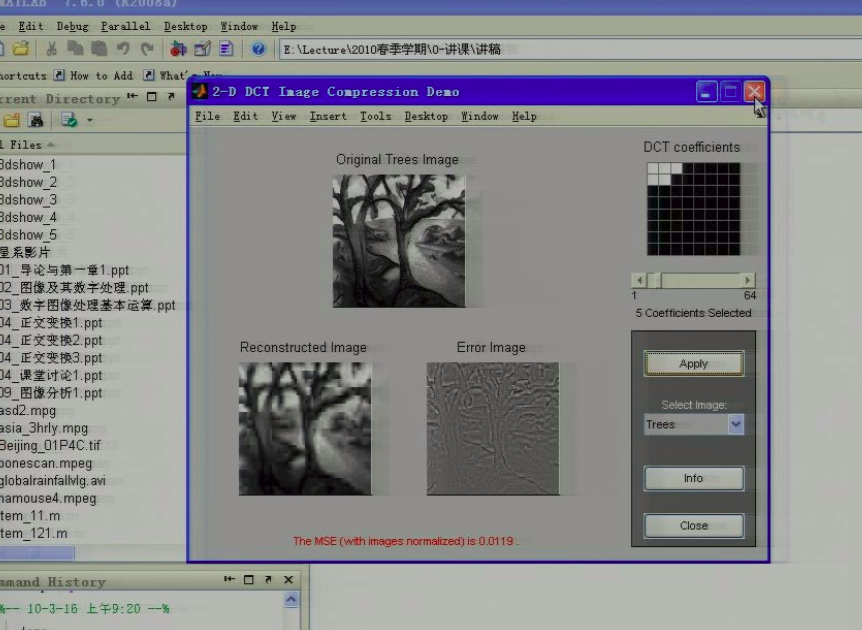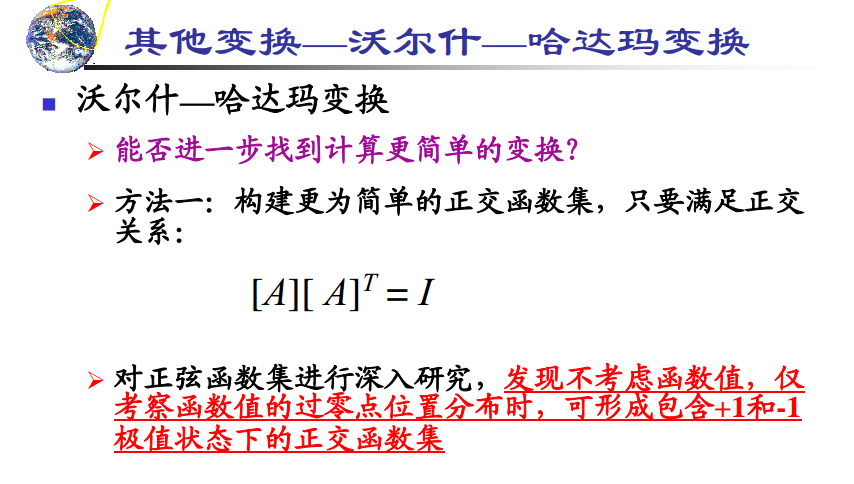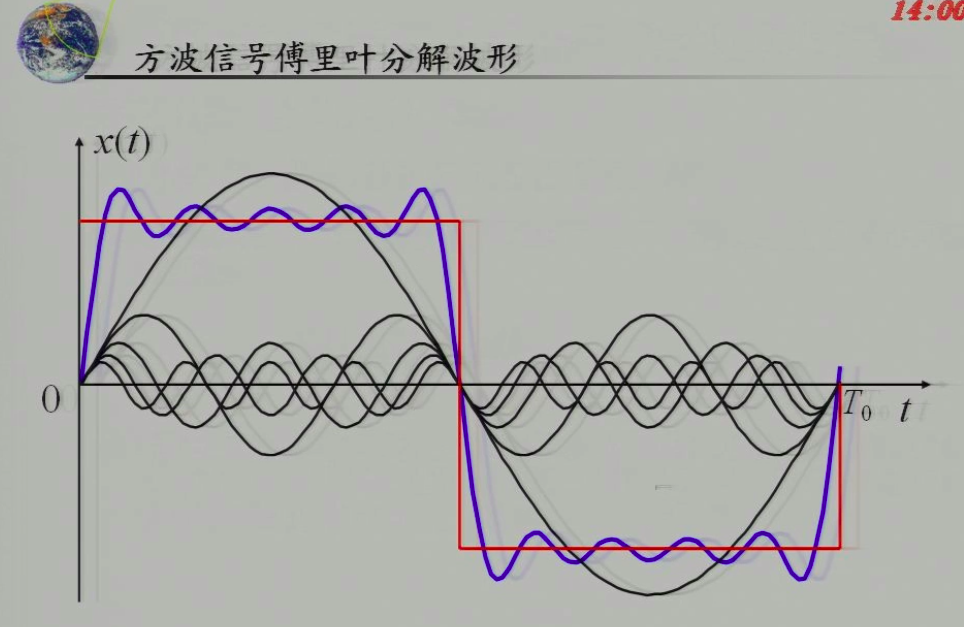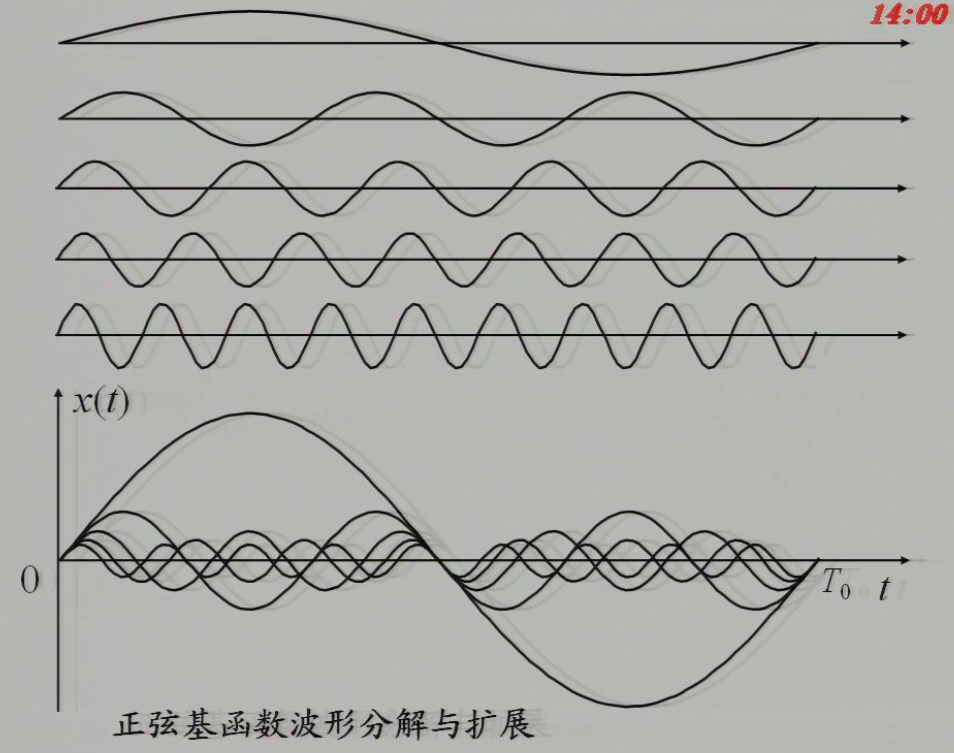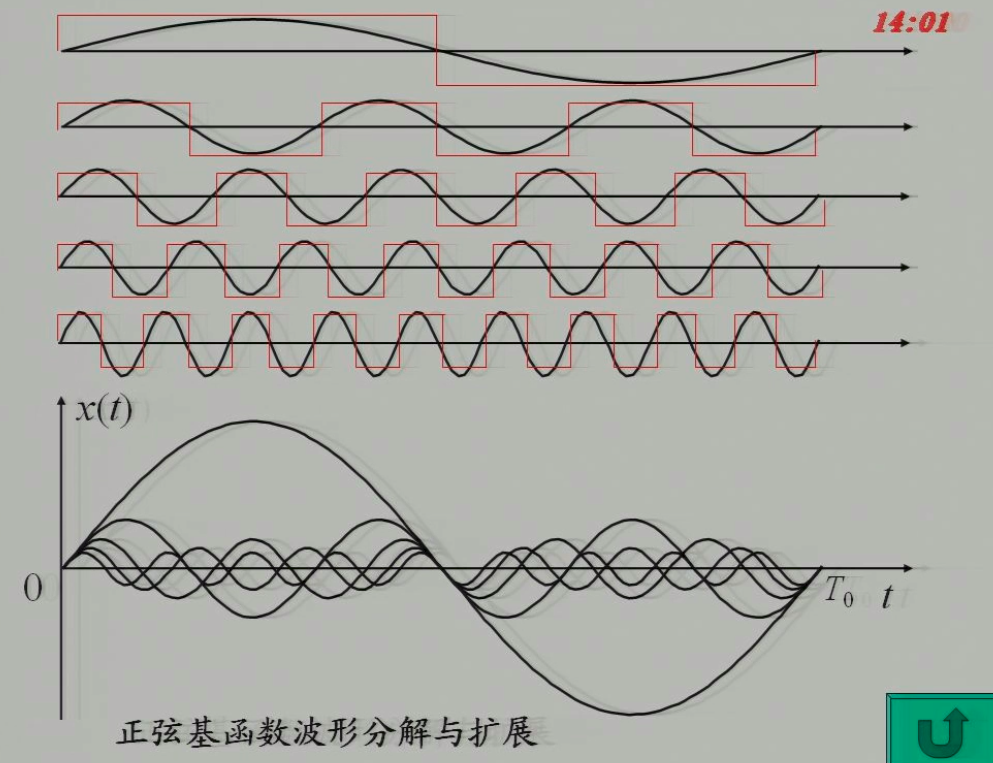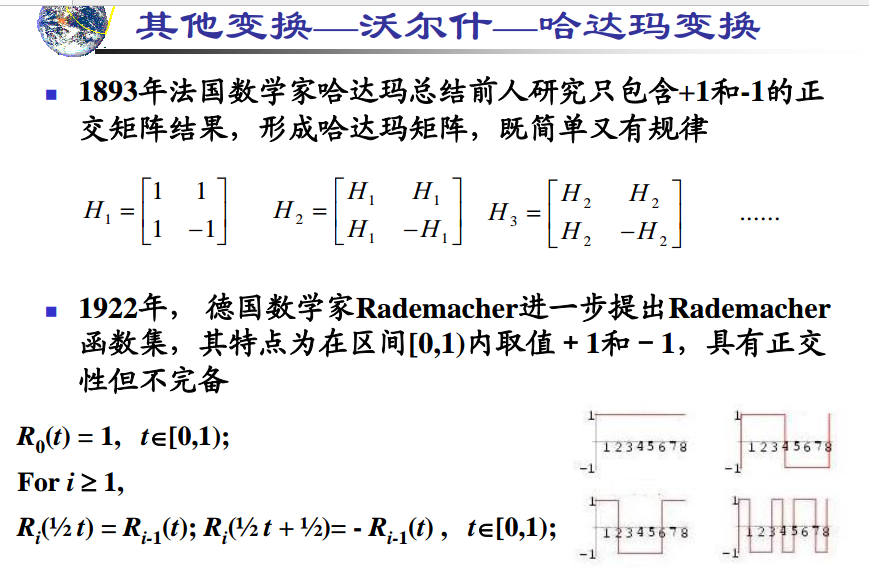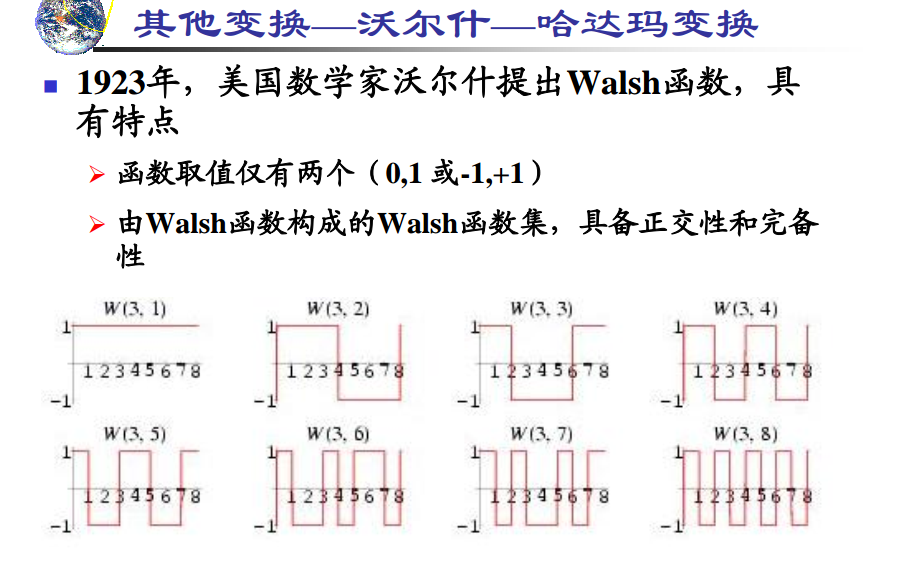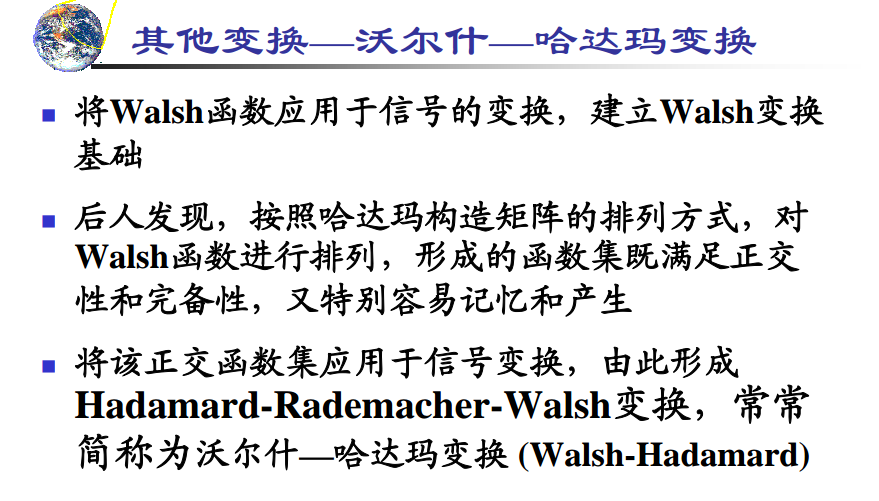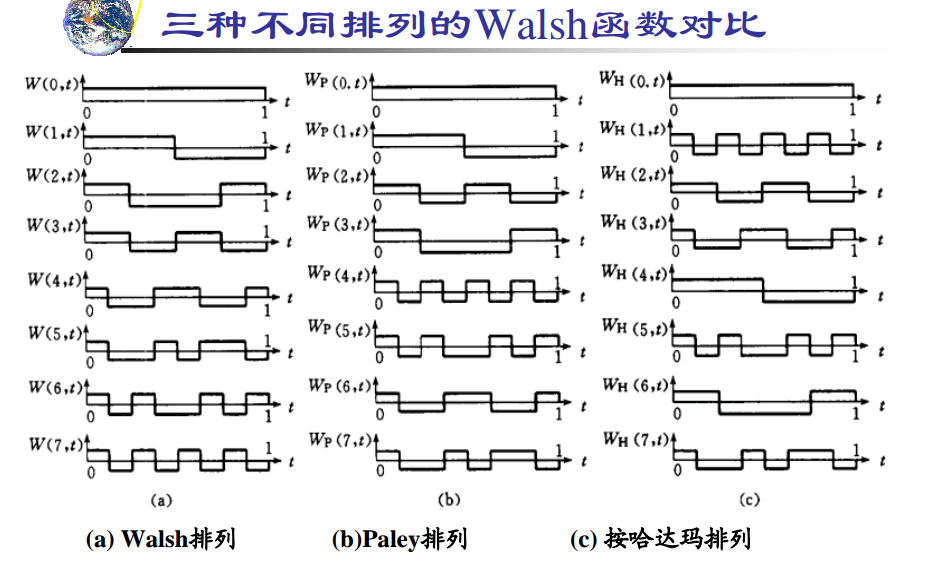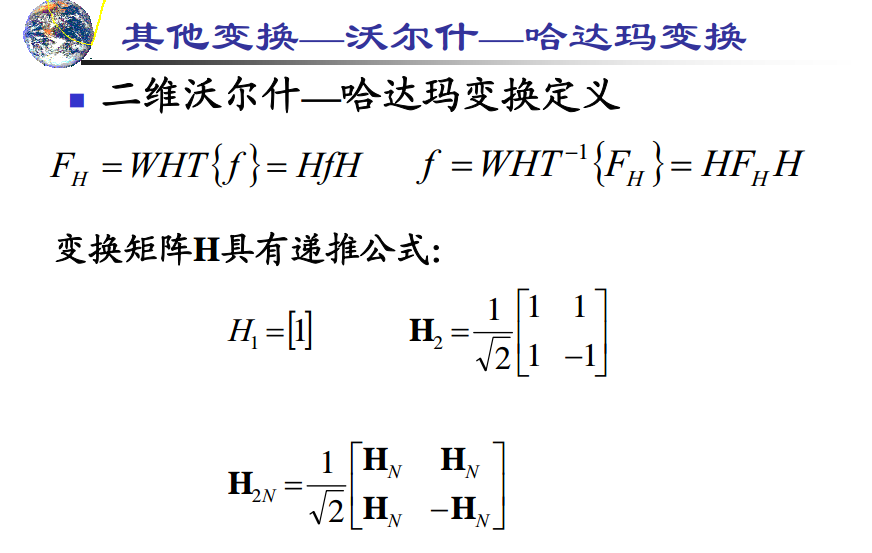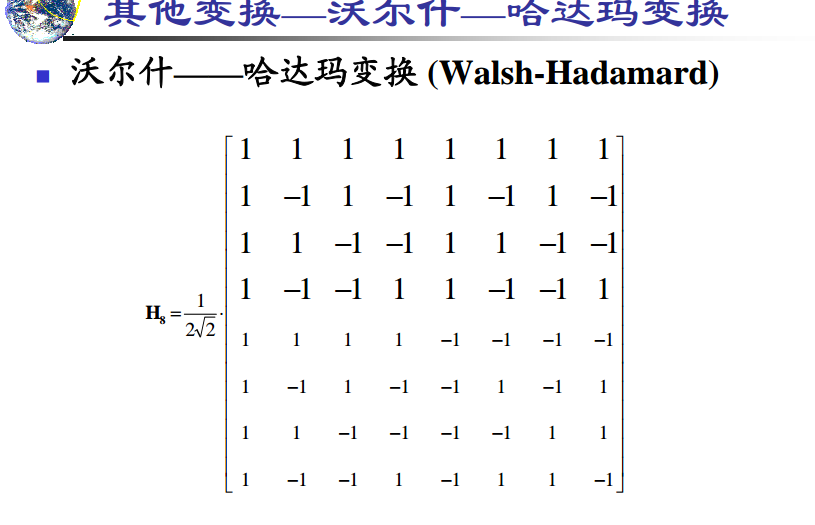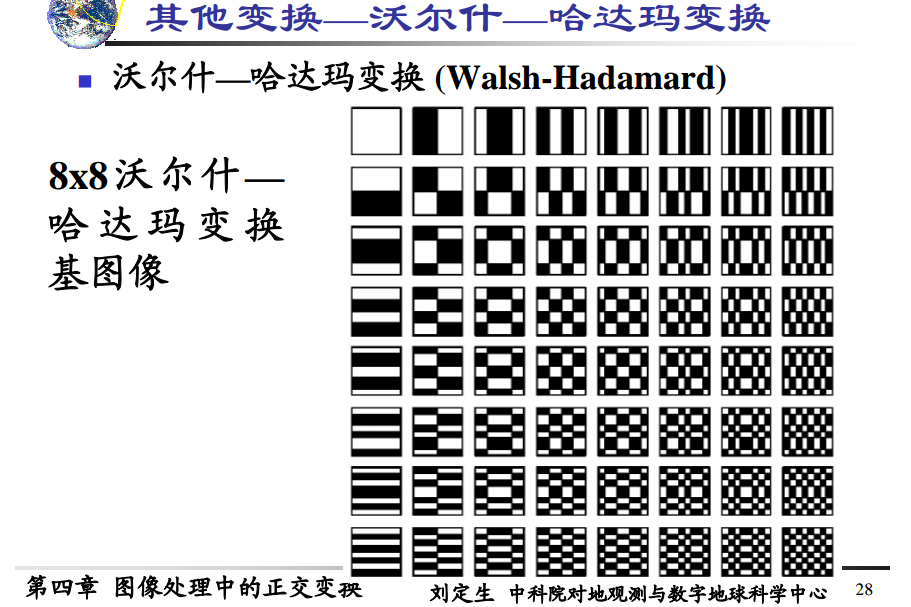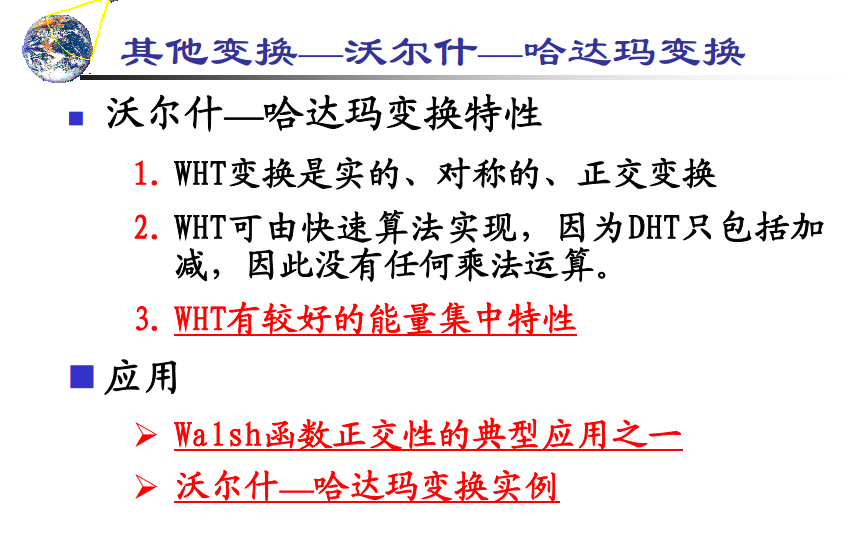哈达玛变换是对离散余弦变换的近似，所以比离散余弦的集中特性要差一点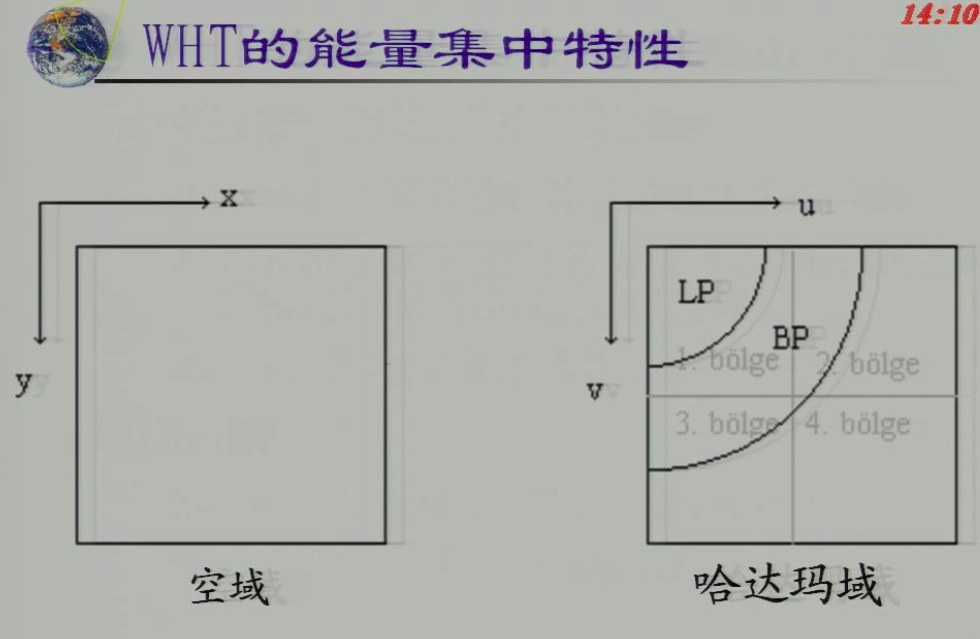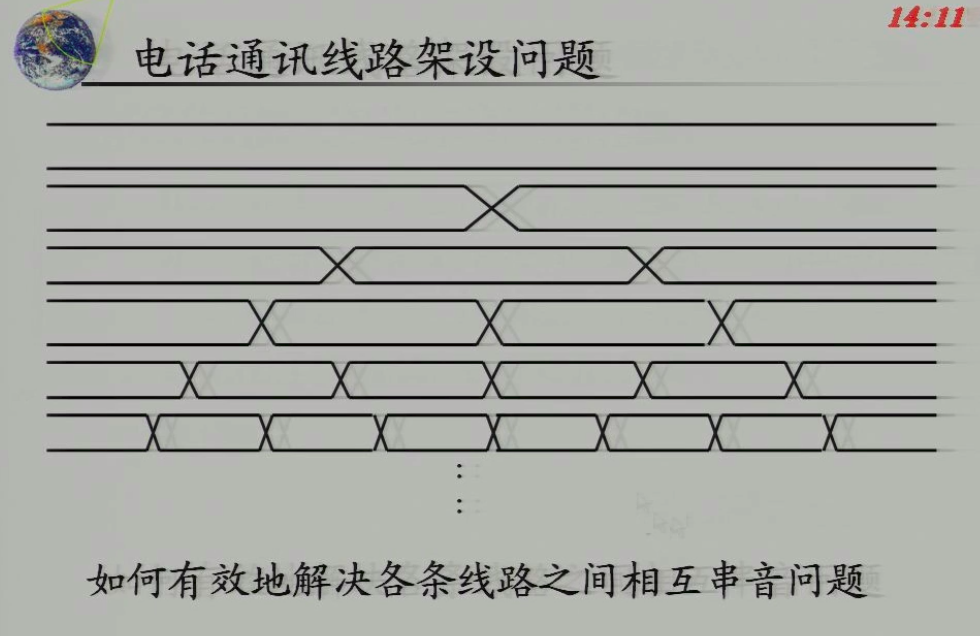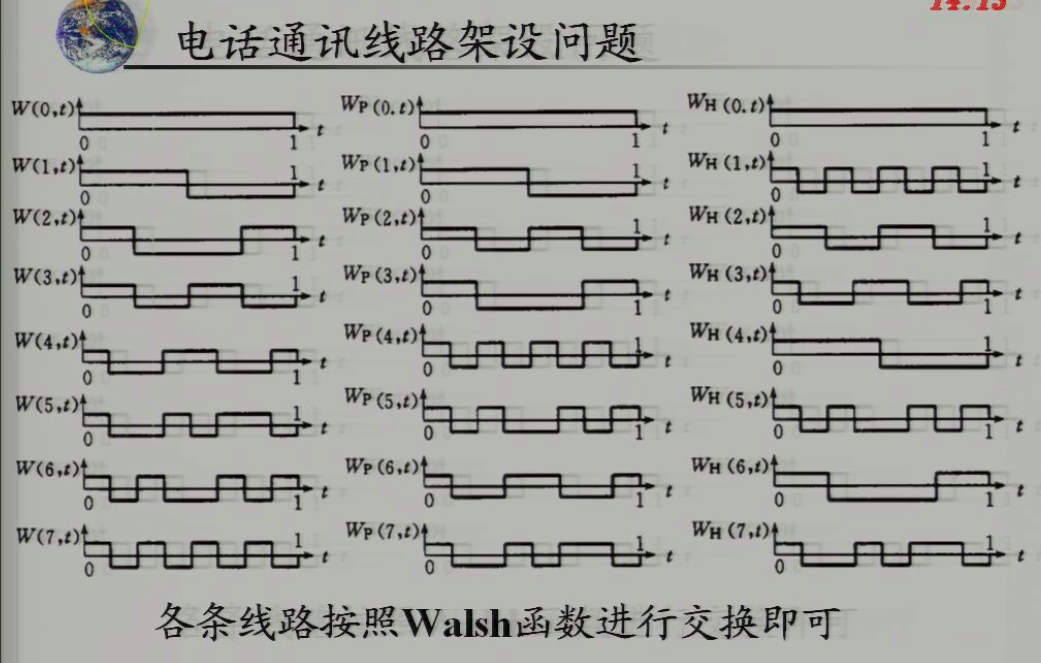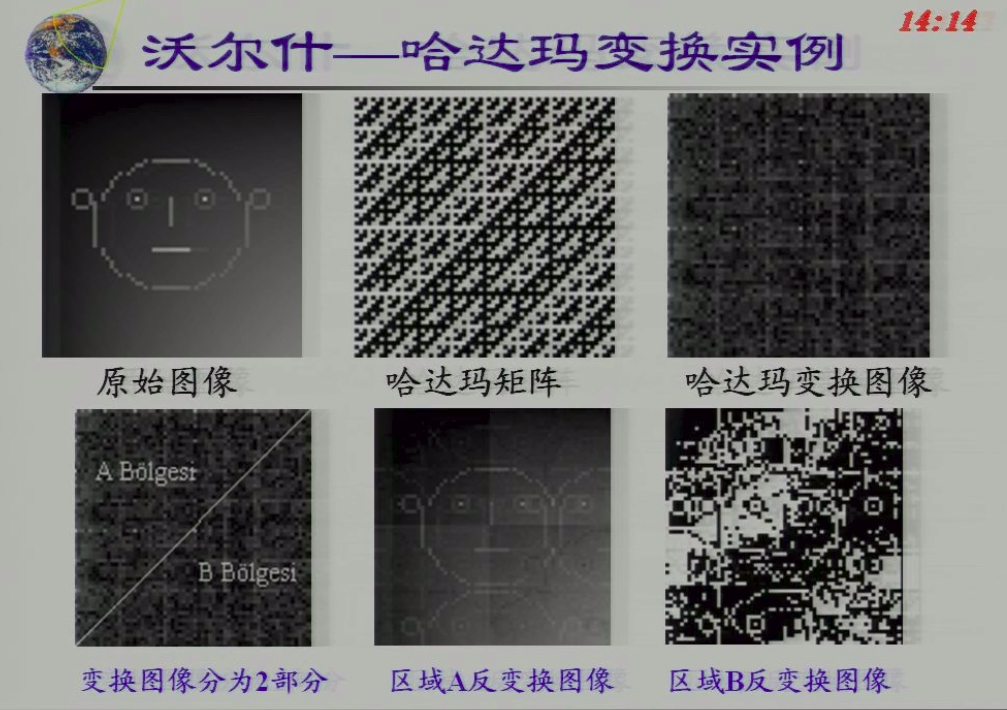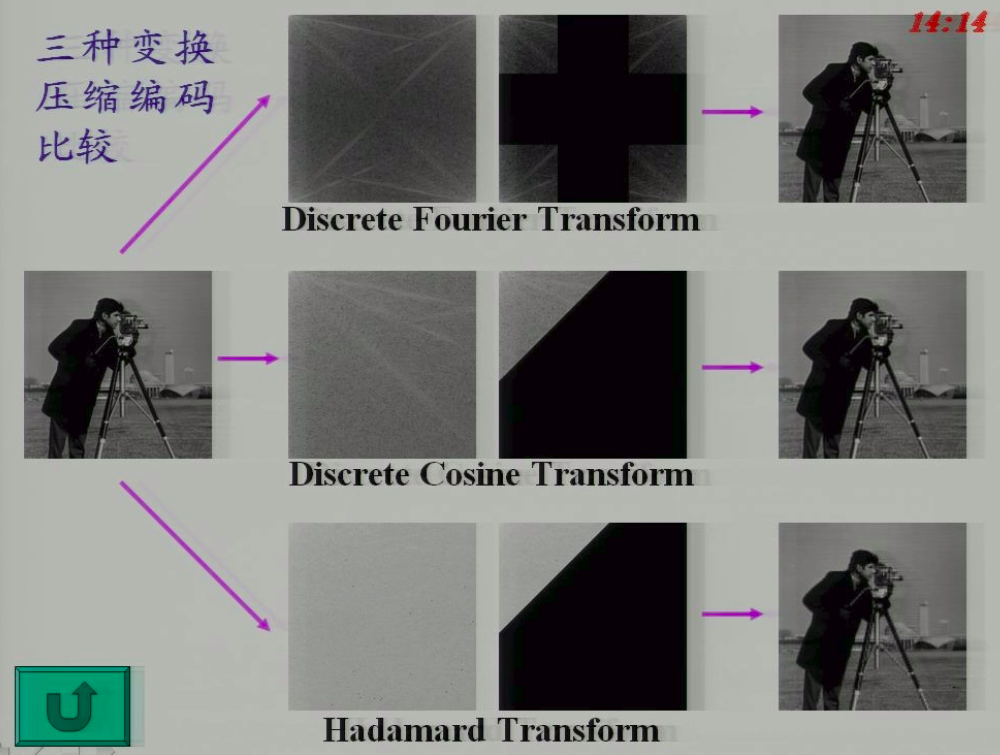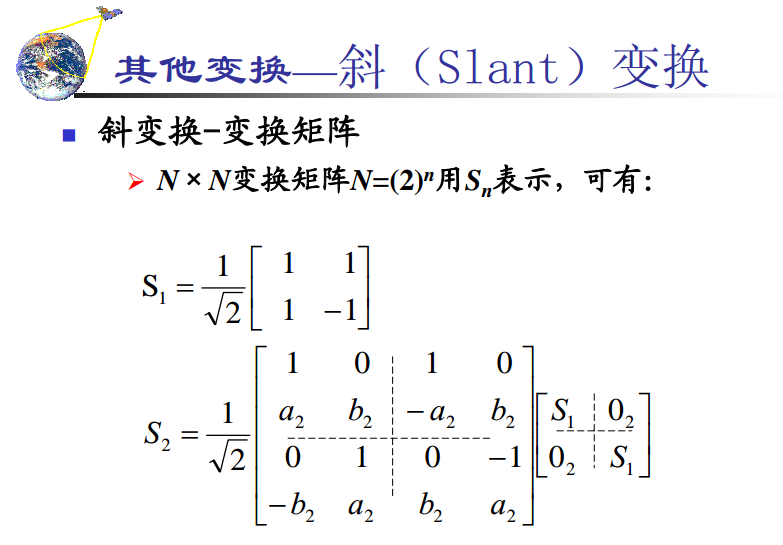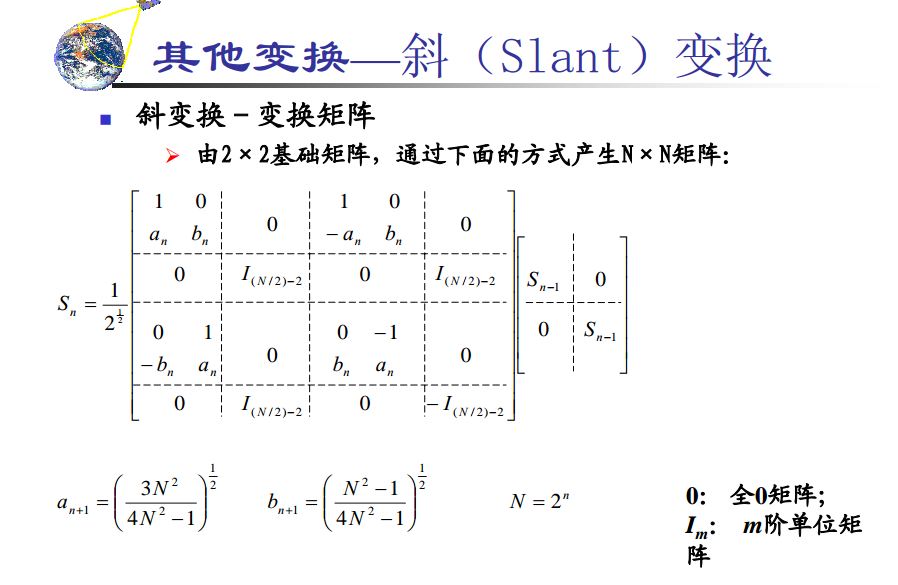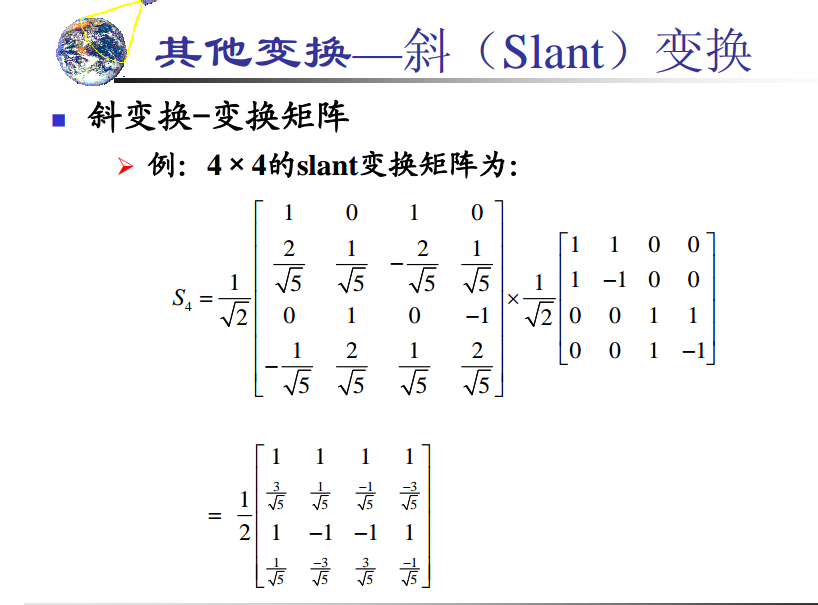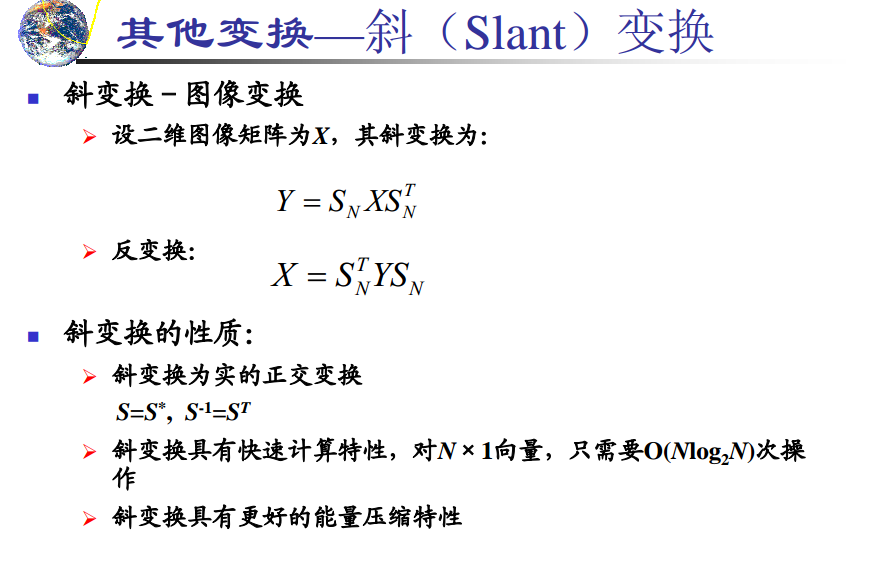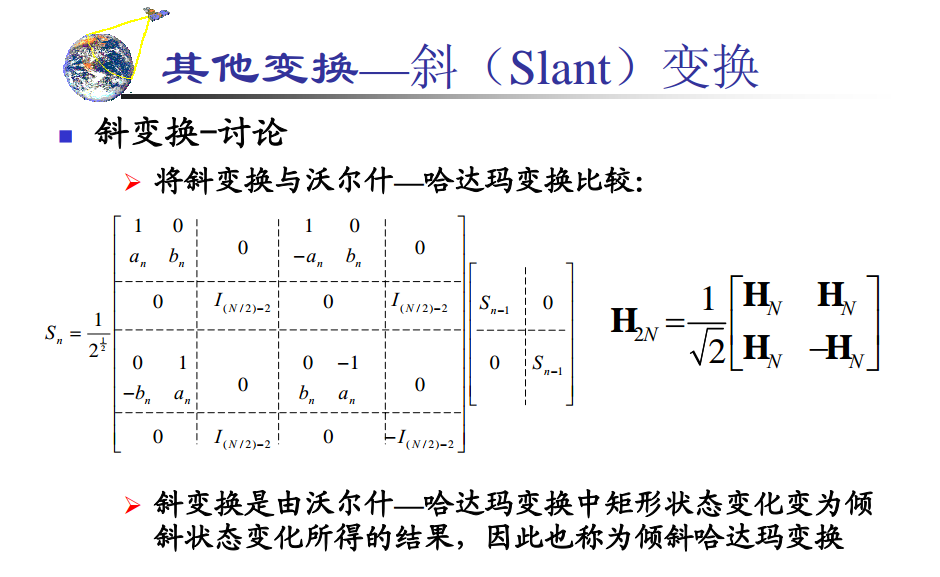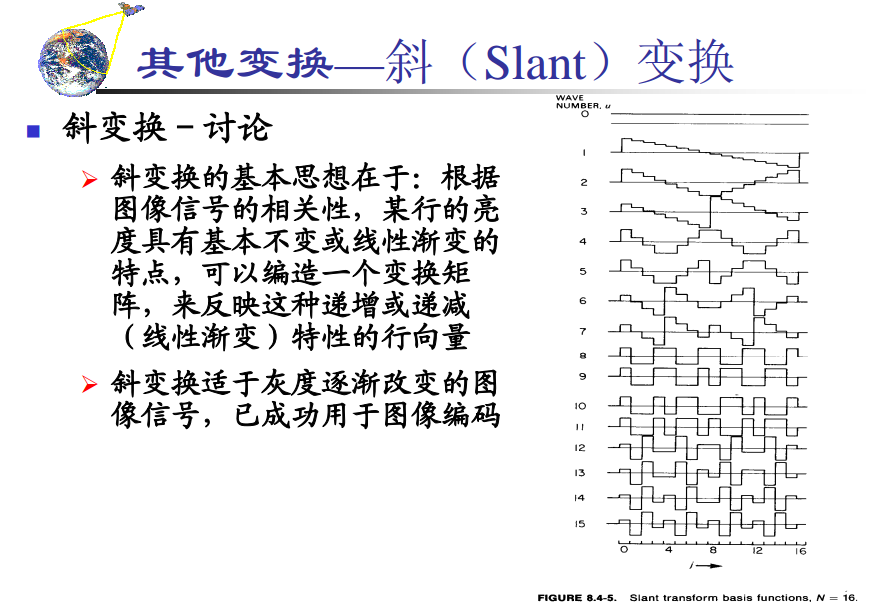展开全文• 哈达玛变换本质上是一种特殊排序的沃尔什变换，哈达玛变换矩阵也是一个方阵，只包括+1和-1两个矩阵元素，各行或各列之间彼此是正交的。哈达玛变换矩阵具有简单的递推关系，即高阶矩阵可用两个低阶矩阵求得。这个特点...图像处理
• 采用哈达玛排列的沃尔什函数进行的变换称为沃尔什-哈达玛变换，简称WHT或直称哈达玛变换沃尔什函数 沃尔什函数有三种不同的函数定义，但都可由拉德梅克函数构成。 1、按沃尔什排列的沃尔什函数 ...


图像处理中有许多变换常常选用方波信号或者它的变形。

沃尔什函数是一组矩形波，其取值为1和-1，非常便于计算机运算。

沃尔什函数有三种排列或编号方式，以哈达玛排列最便于快速计算。

采用哈达玛排列的沃尔什函数进行的变换称为沃尔什-哈达玛变换，简称WHT或直称哈达玛变换。

沃尔什函数

沃尔什函数有三种不同的函数定义，但都可由拉德梅克函数构成。

1、按沃尔什排列的沃尔什函数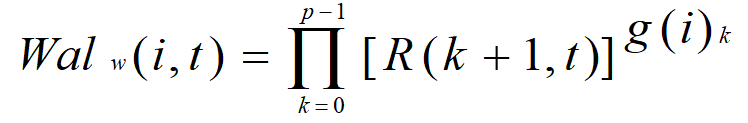其中，R(k+1,t)是任意拉德梅克函数，g(i)是i的格雷码， g(i)k是此格雷码的第k位数。P为正整数，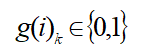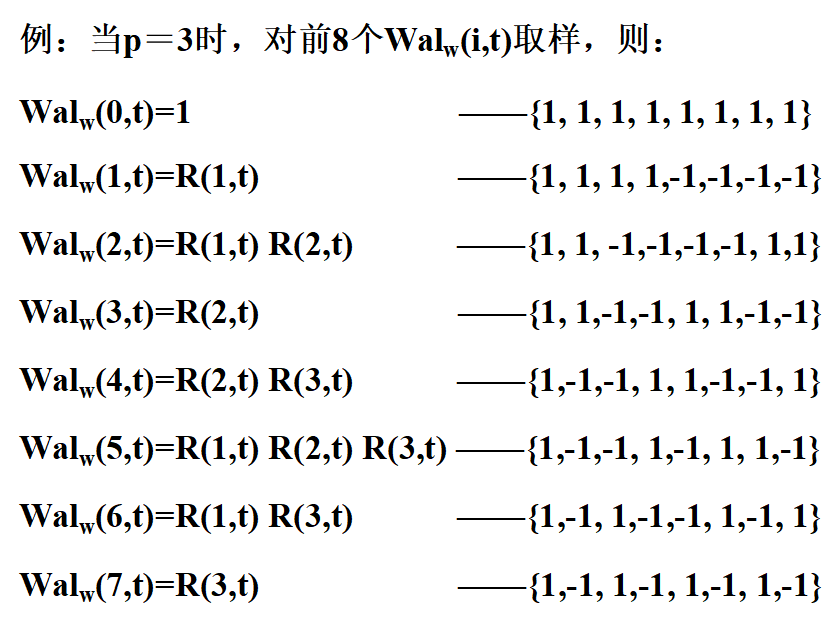取样后得到的按沃尔什排列的沃尔什函数矩阵：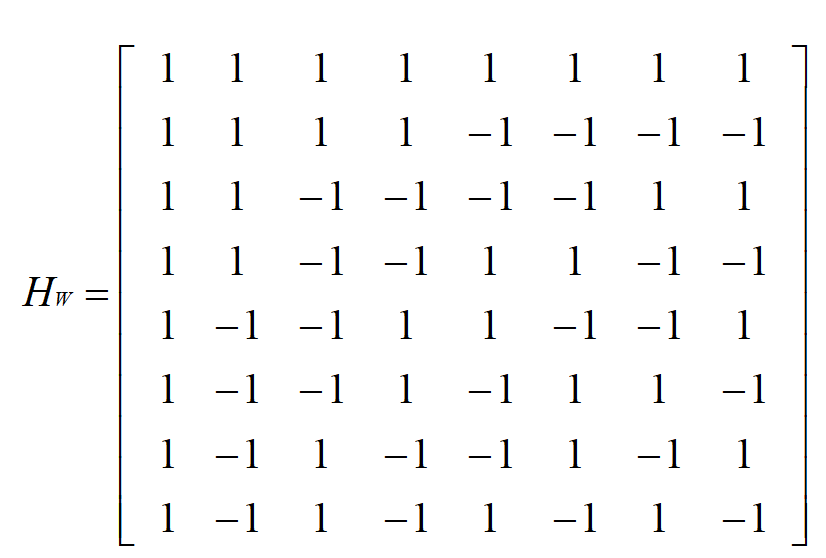2、按佩利（Paley）排列的沃尔什函数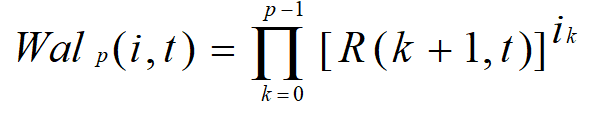其中，R(k+1,t)是任意拉德梅克函数，ik是自然二进制码的第k位数。P为正整数，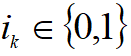。

取样后得到的按佩利排列的沃尔什函数矩阵：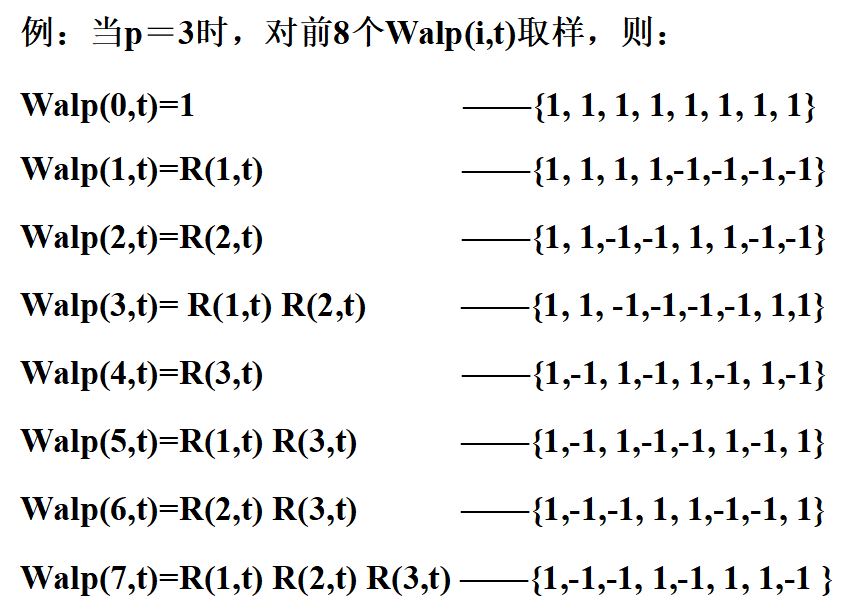3、按哈达玛（Hadamard）排列的沃尔什函数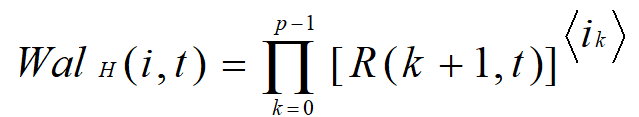其中，R(k+1,t)是任意拉德梅克函数，<ik>是倒序的二进制码的第k位数。P为正整数，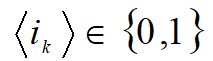。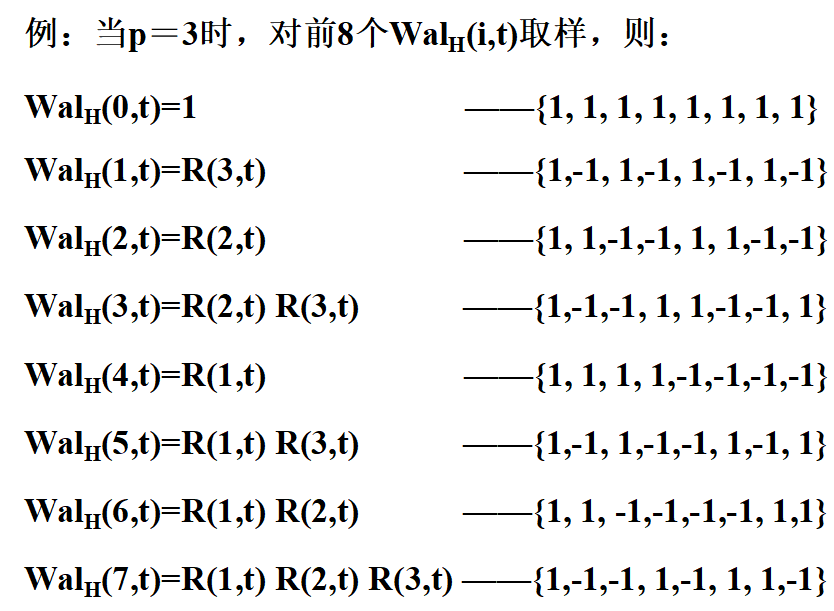取样后得到的按哈达玛排列的沃尔什函数矩阵：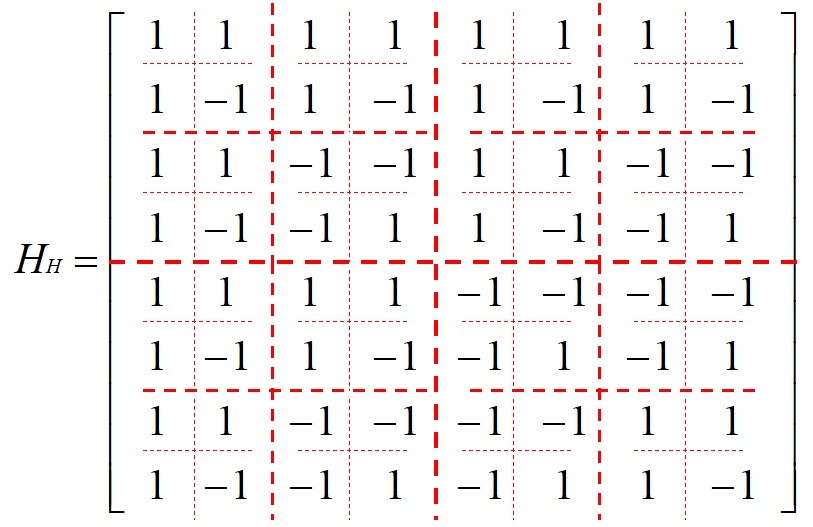2^n阶哈达玛矩阵有如下形式：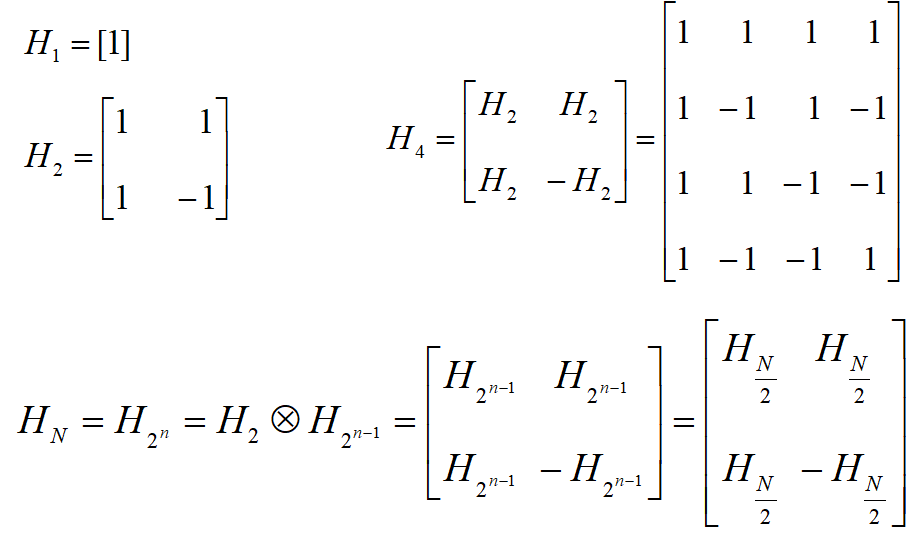可见，哈达玛矩阵的最大优点在于它具有简单的递推关系， 即高阶矩阵可用两个低阶矩阵的克罗内克积(Kronecker Product)求得。因此常采用哈达玛排列定义的沃尔什变换。

离散沃尔什－哈达玛变换（DWHT）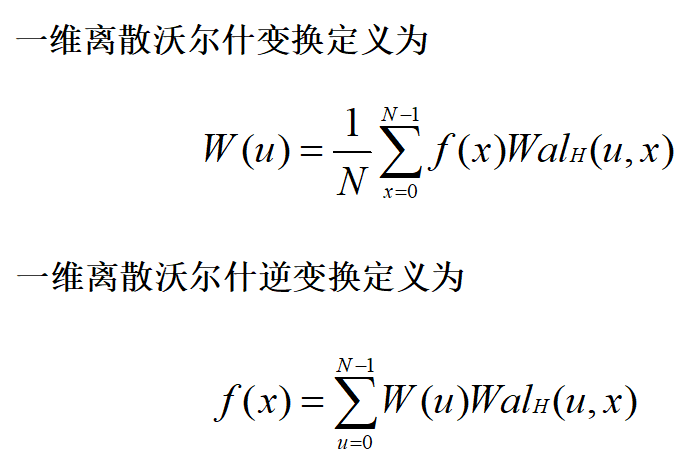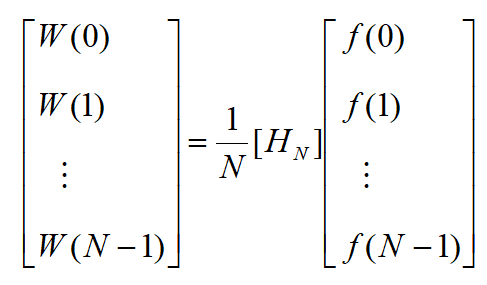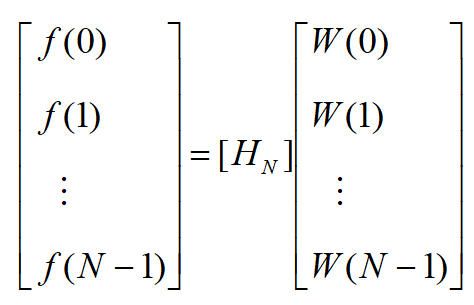式中，［Hn］为N阶哈达玛矩阵。

由哈达玛矩阵的特点可知，沃尔什-哈达玛变换的本质上是将离散序列f(x)的各项值的符号按一定规律改变后，进行加减运算， 因此，它比采用复数运算的DFT和采用余弦运算的DCT要简单得多。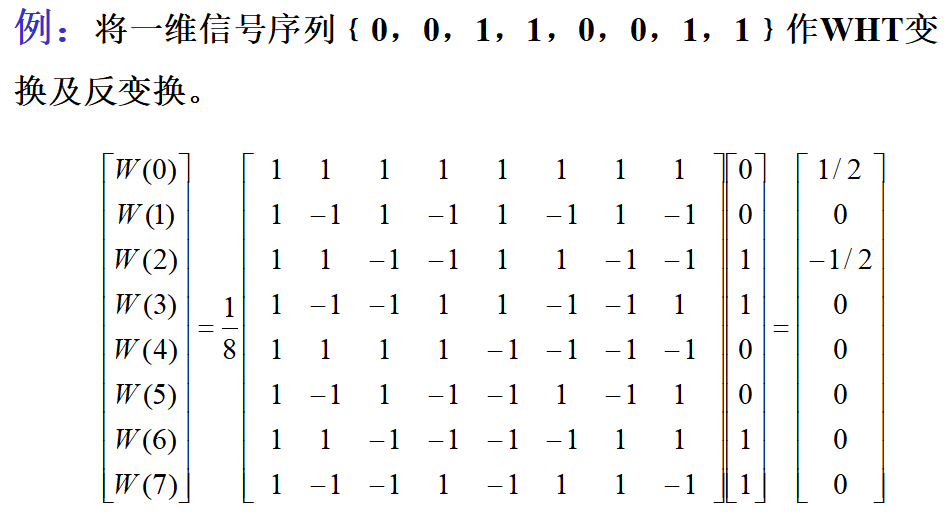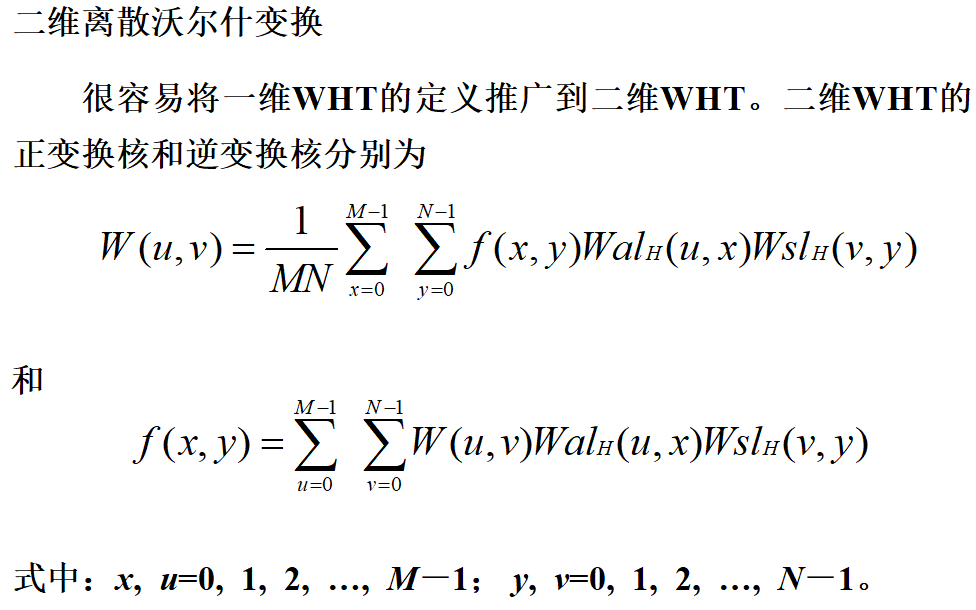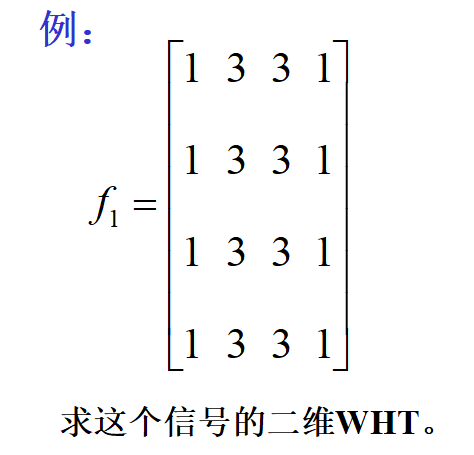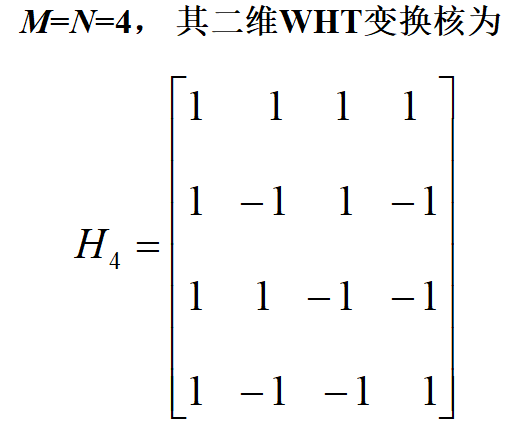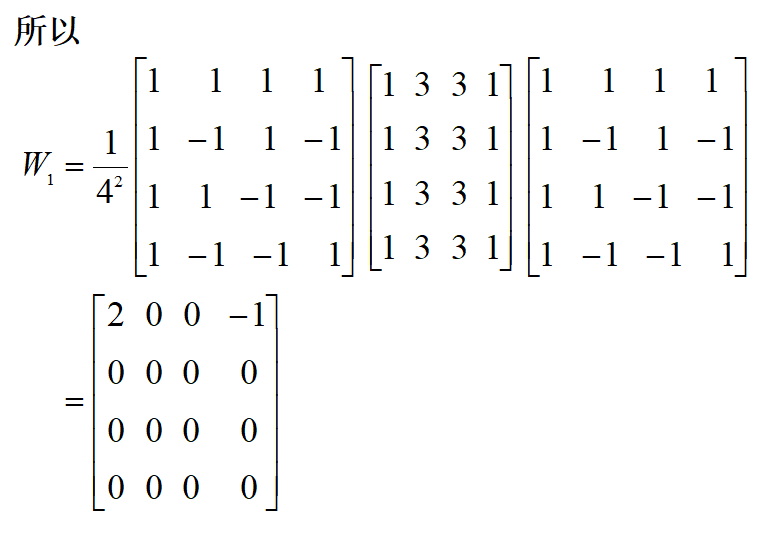从以上例子可看出，二维WHT具有能量集中的特性，而且原始数据中数字越是均匀分布，经变换后的数据越集中于矩阵的边角上。因此，二维WHT可用于压缩图像信息。

快速沃尔什变换（FWHT）

类似于FFT，WHT也有快速算法FWHT， 也可将输入序列f(x)按奇偶进行分组，分别进行WHT。FWHT的基本关系为：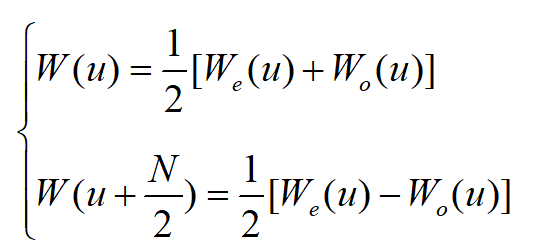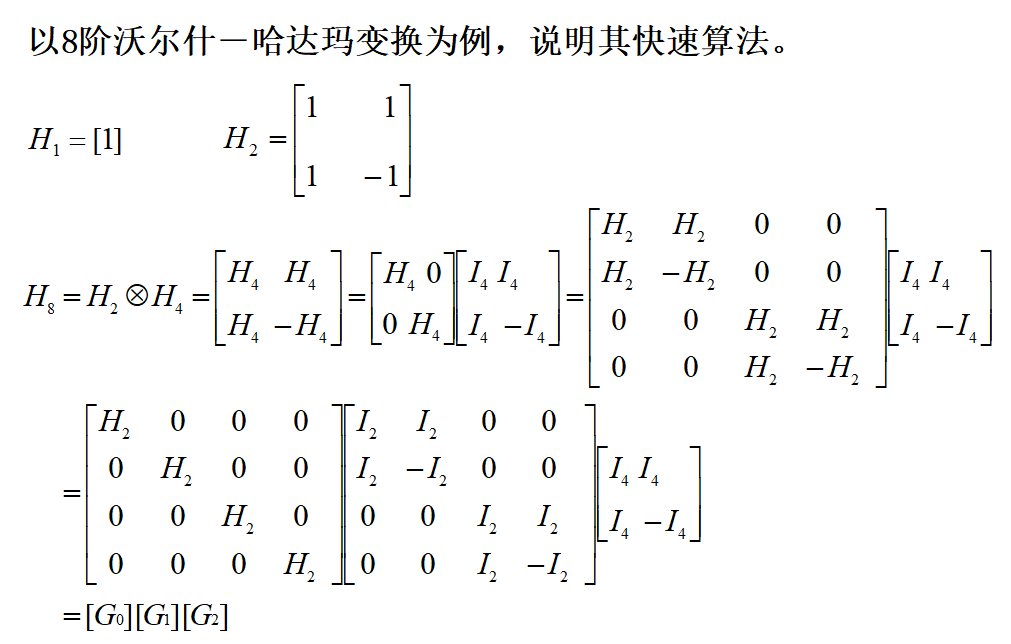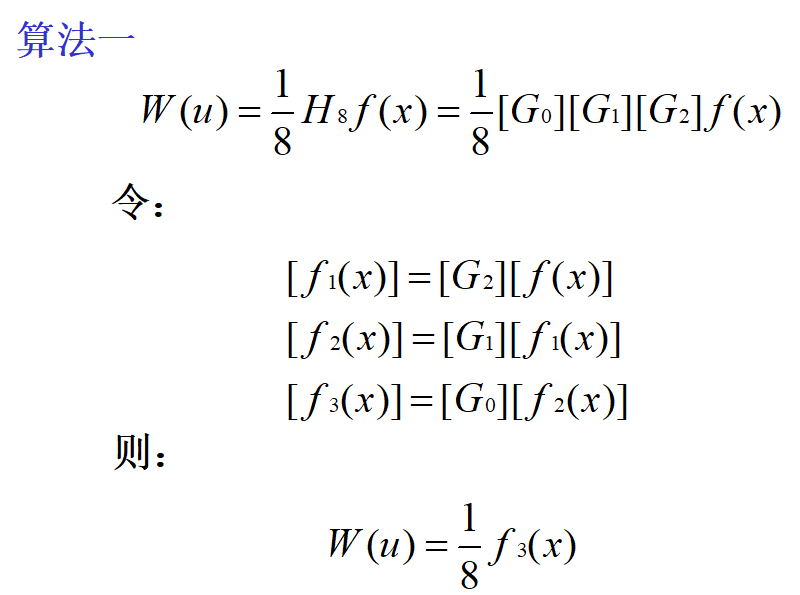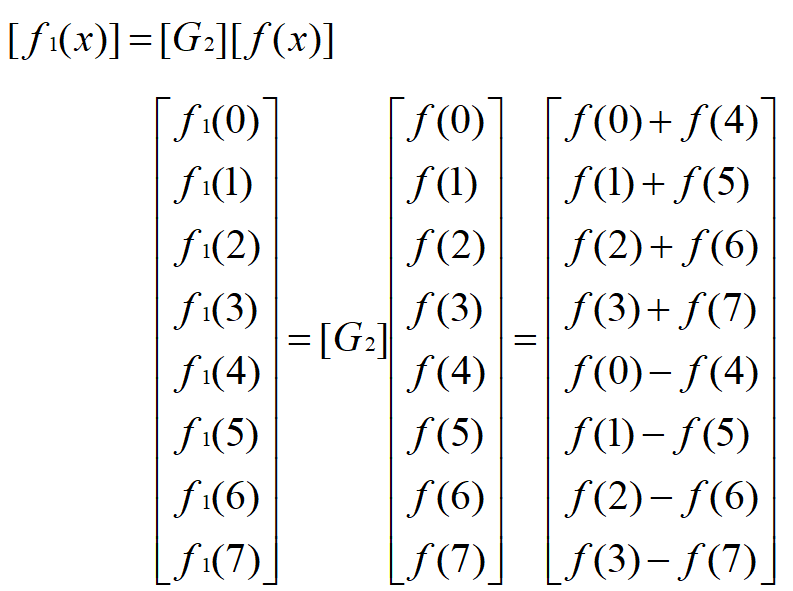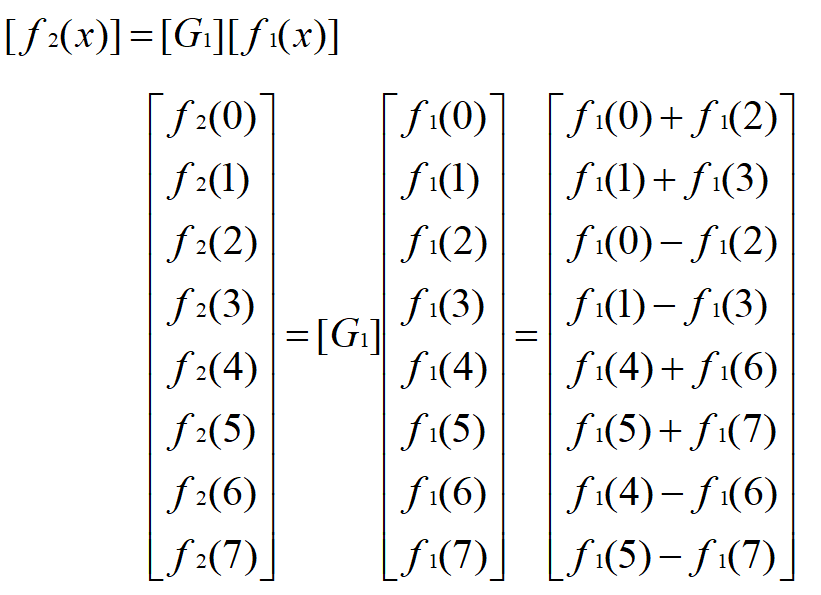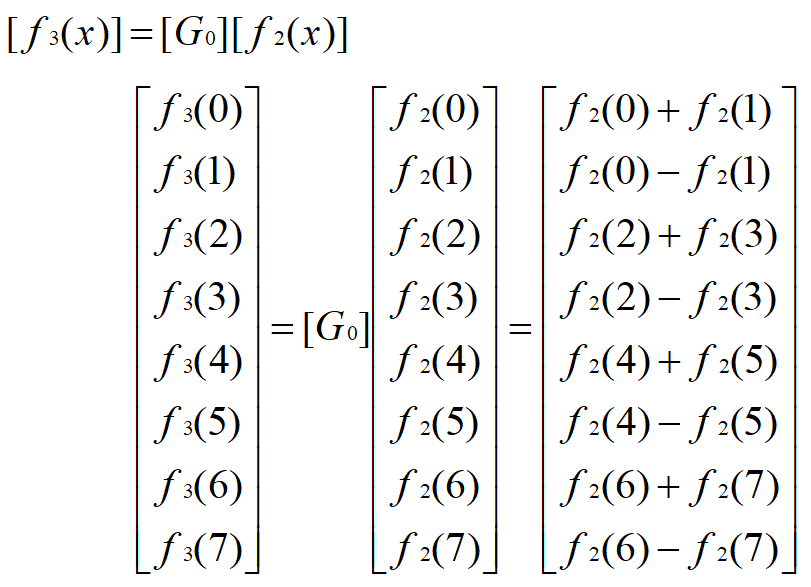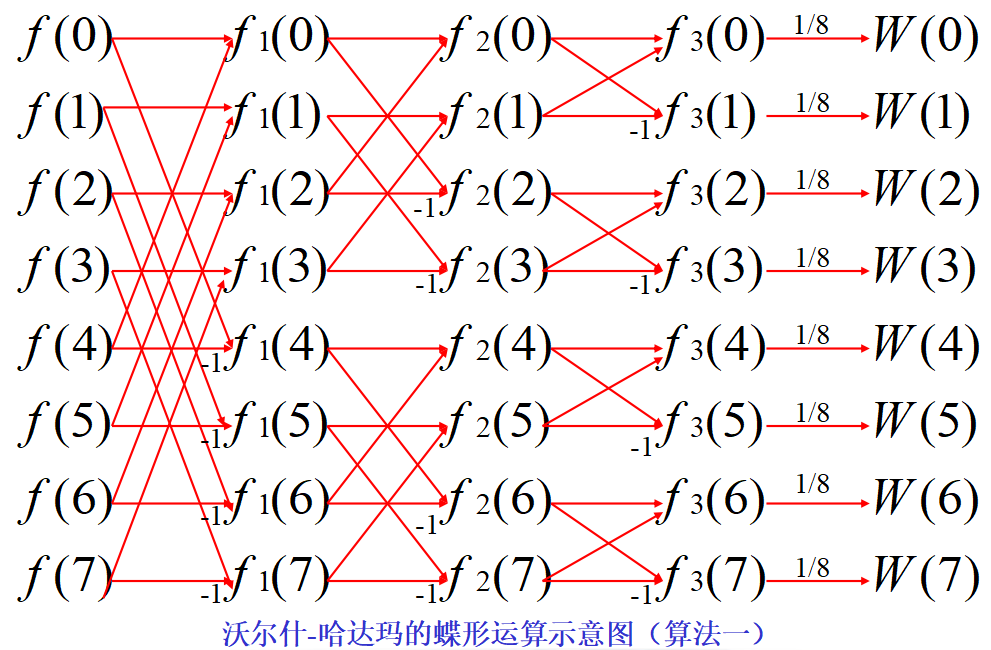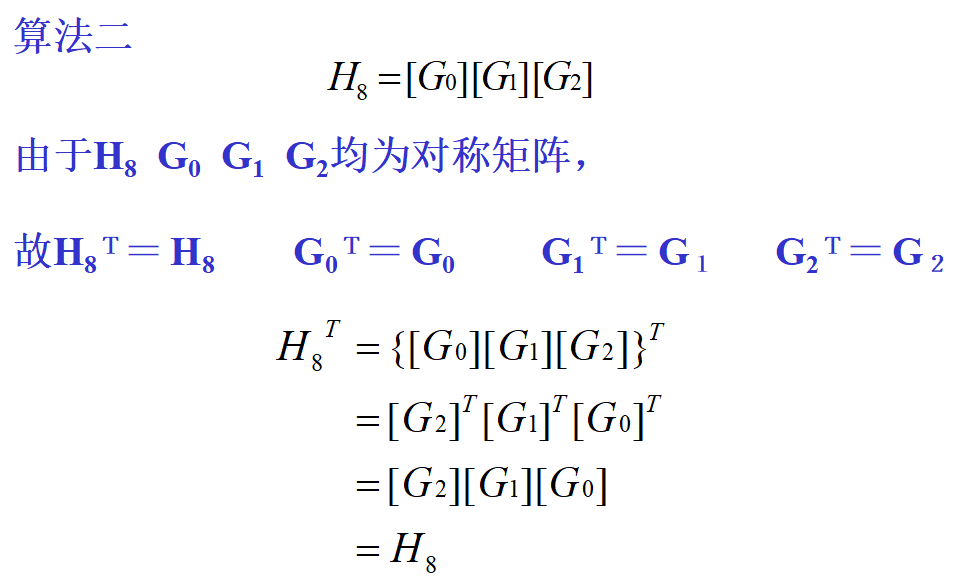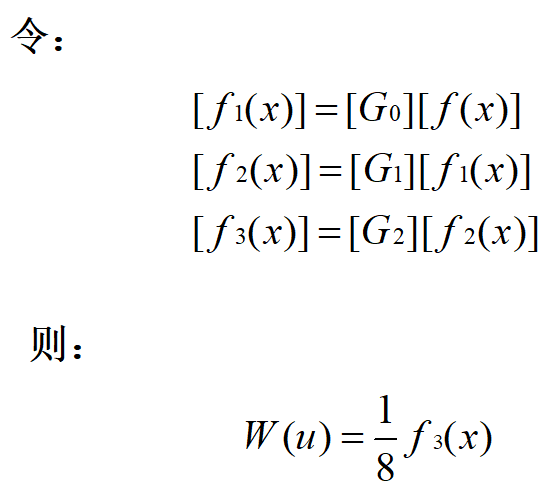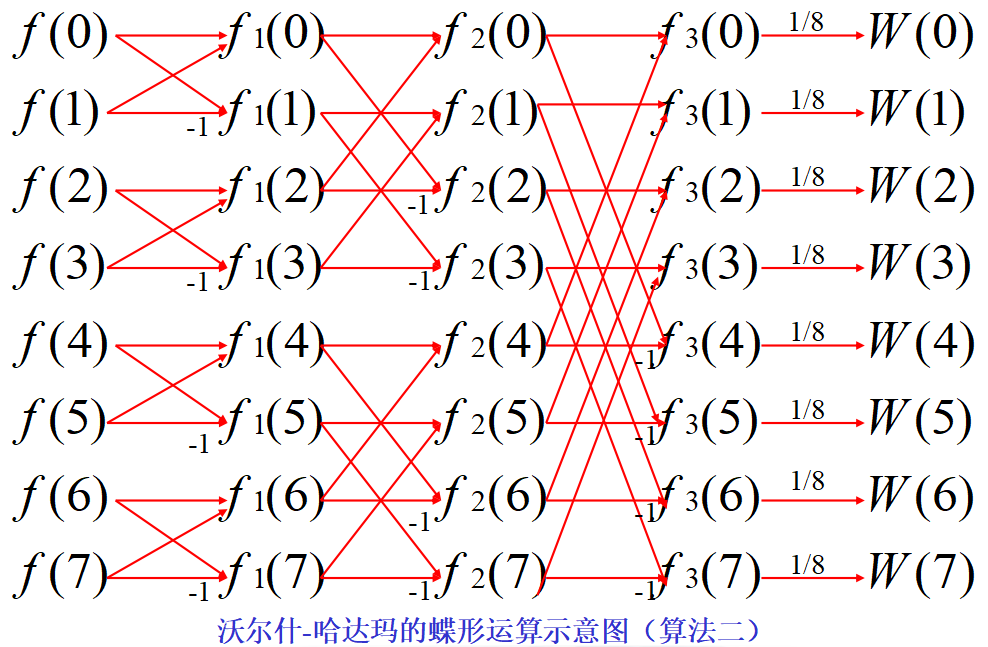WHT是将一个函数变换成取值为＋1或－1的基本函数构成的级数，用它来逼近数字脉冲信号时要比FFT有利。同时， WHT只需要进行实数运算，存储量比FFT要少得多， 运算速度也快得多。因此，WHT在图像传输、 通信技术和数据压缩中被广泛使用。


展开全文• 06-图像的离散沃尔什-哈达玛变换-3.pdf06-图像的离散沃尔什-哈达玛变换-3.pdf
• 由于沃尔什函数是二值正交函数，与数字逻辑中的两个状态相对应，因此它更适用于计算机技术、数字信号处理一维沃尔什变换N=2nN=2^nN=2n哈达玛变换沃尔什变换类似，由哈达玛变换核组成的矩阵是一个正交对称矩阵，...
沃尔什变换
沃尔什变换是由+1或-1的基本函数的级数展开而成的，满足完备正交特性，属于方波型正交变换。由于沃尔什函数是二值正交函数，与数字逻辑中的两个状态相对应，因此它更适用于计算机技术、数字信号处理
一维沃尔什变换
沃尔什变换要求空域矩阵阶数$N$满足$N=2^n$，若满足，则有：$F(\mu)=\sum_{x=0}^{N-1}f(x)g(x,\mu)$$g(x,\mu)=\frac{1}{N}\prod_{i=0}^{n-1}(-1)^{\sum_{i=0}^{n-1}b_i(x)b_{n-1}(\mu)}$一维沃尔什反变换：$f(x)=\sum_{x=0}^{N-1}F(\mu)h(x,\mu)$$h(x,\mu)=\prod_{i=0}^{n-1}(-1)^{\sum_{i=0}^{n-1}b_i(x)b_{n-1}(\mu)}$其中，$b_i(x)$是$x$的二进制表达式中第$i$位的意思
例如$N=2^n$，$n=3$，$x=6$，则有$x=6=(110)_2$，可得$b_0(x)=0$、$b_1(x)=1$、$b_2(x)=1$
与傅立叶变换不同，沃尔什变换的变换核是由+1或-1构成的二值对称矩阵
例如：求$N=2^2$的沃尔什变换$F(0)=\frac{1}{4}\sum_{x=0}^{3}[f(x)\prod_{i=0}^{1}(-1)^{b_i(x)+b_{1-i}(0)}]=\frac{1}{4}[f(0)+f(1)+f(2)+f(3)]$$F(1)=\frac{1}{4}\sum_{x=0}^{3}[f(x)\prod_{i=0}^{1}(-1)^{b_i(x)+b_{1-i}(1)}]=\frac{1}{4}[f(0)+f(1)-f(2)-f(3)]$$F(2)=\frac{1}{4}\sum_{x=0}^{3}[f(x)\prod_{i=0}^{1}(-1)^{b_i(x)+b_{1-i}(2)}]=\frac{1}{4}[f(0)-f(1)+f(2)-f(3)]$$F(3)=\frac{1}{4}\sum_{x=0}^{3}[f(x)\prod_{i=0}^{1}(-1)^{b_i(x)+b_{1-i}(3)}]=\frac{1}{4}[f(0)-f(1)-f(2)+f(3)]$其中方括号中各项的符号即位变换核的符号
可见沃尔什变换的本质是将离散序列$f(x)$中各项值的符号按规律改变进行加减运算
二维沃尔什变换
$F(\mu,\nu)=\frac{1}{N}\sum_{x=0}^{N-1}\sum_{x=0}^{N-1}f(x,y)g(x,y,\mu,\nu)$$f(x,y)=\frac{1}{N}\sum_{\mu=0}^{N-1}\sum_{\nu=0}^{N-1}F(\mu,\nu)g(x,y,\mu,\nu)$$g(x,y,\mu,\nu)=G=\frac{1}{N}\prod_{i=0}^{n-1}(-1)^{\sum_{i=0}^{n-1}[b_i(x)b_{n-1-i}(\mu)+b_i(y)b_{n-1-i}(\nu)]}$表达为矩阵形式为$F=\frac{1}{N^2}GfG$$f=GFG$
与傅立叶变换一样，二维沃尔什变换是可分离的，可以通过两个一维沃尔什变换完成计算$g(x,y,\mu,\nu)=g_1(x,\mu)g_2(y,\nu)$
实例：求离散沃尔什变换
$f=\begin{bmatrix} 1 & 3 & 3 & 1 \\ 1 & 3 & 3 & 1 \\ 1 & 3 & 3 & 1 \\ 1 & 3 & 3 & 1 \\ \end{bmatrix}$可知$N=4$，其变换核为$G=\begin{bmatrix} 1 & 1 & 1 & 1 \\ 1 & 1 & -1 & -1 \\ 1 & -1 & 1 & -1 \\ 1 & -1 & -1 & 1 \\ \end{bmatrix}$则有$F=\frac{1}{N^2}GfG=\frac{1}{4^2}\begin{bmatrix} 1 & 1 & 1 & 1 \\ 1 & 1 & -1 & -1 \\ 1 & -1 & 1 & -1 \\ 1 & -1 & -1 & 1 \\ \end{bmatrix}\begin{bmatrix} 1 & 3 & 3 & 1 \\ 1 & 3 & 3 & 1 \\ 1 & 3 & 3 & 1 \\ 1 & 3 & 3 & 1 \\ \end{bmatrix}\begin{bmatrix} 1 & 1 & 1 & 1 \\ 1 & 1 & -1 & -1 \\ 1 & -1 & 1 & -1 \\ 1 & -1 & -1 & 1 \\ \end{bmatrix}=\begin{bmatrix} 2 & 0 & 0 & -1 \\ 0 & 0 & 0 & 0 \\ 0 & 0 & 0 & 0 \\ 0 & 0 & 0 & 0 \\ \end{bmatrix}$可以看出，沃尔什变换具有信息集中的作用，原始数据中数字越是均匀分布，变换后的数据越是集中于边角区域
哈达玛变换
与沃尔什变换类似，由哈达玛变换核组成的矩阵是一个正交对称矩阵，属于方波型正交变换，不同之处在于其行、列次序不一样。
一维哈达玛变换
哈达玛变换要求空域矩阵阶数$N$满足$N=2^n$，若满足，则有：$F(\mu)=\sum_{x=0}^{N-1}f(x)g(x,\mu)$$g(x,\mu)=\frac{1}{N}(-1)^{\sum_{i=0}^{n-1}b_i(x)b_{i}(\mu)}$一维哈达玛反变换：$f(x)=\sum_{x=0}^{N-1}F(\mu)h(x,\mu)$$h(x,\mu)=(-1)^{\sum_{i=0}^{n-1}b_i(x)b_{i}(\mu)}$$b_i(x)$是$x$的二进制表达式中第$i$位的意思
二维哈达玛变换
$F(\mu,\nu)=\frac{1}{N}\sum_{x=0}^{N-1}\sum_{x=0}^{N-1}f(x,y)g(x,y,\mu,\nu)$$f(x,y)=\frac{1}{N}\sum_{\mu=0}^{N-1}\sum_{\nu=0}^{N-1}F(\mu,\nu)g(x,y,\mu,\nu)$$g(x,y,\mu,\nu)=G=\frac{1}{N}(-1)^{\sum_{i=0}^{n-1}[b_i(x)b_{i}(\mu)+b_i(y)b_{i}(\nu)]}$二维哈达玛变换是可分离的，可变换为两个一维哈达玛变换来进行计算。
列率
在哈达玛矩阵中，沿列方向上符号改变的次数称为列率，类似傅立叶变换中频率的概念。
例如有矩阵$H=\begin{bmatrix} 1 & 1 & 1 & 1 \\ 1 & -1 & 1 & -1\\ 1 & 1 & -1 & -1 \\ 1 & -1 & -1 & 1\\ \end{bmatrix}$
其第一列元素符号无变化，于是第一列的列率为0；
第二列$a_{12}$到$a_{22}$符号由正变负、$a_{22}$到$a_{32}$符号由负变正、$a_{32}$到$a_{42}$符号由正变负，共改变3次，因此第二列的列率为3；以此类推，第三列的列率为1，第四列的列率为2
哈达玛变换在列率上是随机的，不利于实现逐次倍加法的快速运算，但是能导出一个简单的递推关系，构造其变换矩阵，而后进行哈达玛正向和反向变换
哈达玛变换核矩阵
在满足$N=2^n$的情况下
有最低阶$N=2$时的哈达玛变换矩阵$H_2$为：$H_2=\begin{bmatrix} 1 & 1 \\ 1 & -1 \\ \end{bmatrix}$有任意阶$N=2N$时的哈达玛矩阵为：$H_{2N}=\begin{bmatrix} H_N & H_N \\ H_N & -H_N \\ \end{bmatrix}$由此可推得：$H_{4}=\begin{bmatrix} H_2 & H_2 \\ H_2 & -H_2 \\ \end{bmatrix},H_{8}=\begin{bmatrix} H_4 & H_4 \\ H_4 & -H_4 \\ \end{bmatrix},...$
将哈达玛矩阵按照列率顺序排列，得到定序的哈达玛变换对为：$F(\mu,\nu)=\frac{1}{N}\sum_{x=0}^{N-1}\sum_{x=0}^{N-1}f(x,y)g(x,y,\mu,\nu)$$f(x,y)=\frac{1}{N}\sum_{\mu=0}^{N-1}\sum_{\nu=0}^{N-1}F(\mu,\nu)g(x,y,\mu,\nu)$$g(x,y,\mu,\nu)=G=\frac{1}{N}(-1)^{\sum_{i=0}^{n-1}[b_i(x)p_{i}(\mu)+b_i(y)p_{i}(\nu)]}$其中\begin{aligned} p_0(\mu) & = b_{n-1}(\mu) \\ p_i(\mu) & = b_{n-i}(\mu)+b_{n-1-i}(\mu) \\ \end{aligned}表达为矩阵形式为$F=\frac{1}{N^2}HfH$$f=HFH$
实例：求离散哈达玛变换$f=\begin{bmatrix} 1 & 3 & 3 & 1 \\ 1 & 3 & 3 & 1 \\ 1 & 3 & 3 & 1 \\ 1 & 3 & 3 & 1 \\ \end{bmatrix}$易知$N=4$，其哈达玛变换核矩阵为：$H_{4}=\begin{bmatrix} H_2 & H_2 \\ H_2 & -H_2 \\ \end{bmatrix}=\begin{bmatrix} 1 & 1 & 1 & 1 \\ 1 & -1 & 1 & -1\\ 1 & 1 & -1 & -1 \\ 1 & -1 & -1 & 1\\ \end{bmatrix}$$H_4$按列率排序后得到$H_4=\begin{bmatrix} 1 & 1 & 1 & 1 \\ 1 & 1 & -1 & -1\\ 1 & -1 & -1 & 1 \\ 1 & -1 & 1 & -1\\ \end{bmatrix}$由此按照哈达玛变换的计算公式可得$F=\frac{1}{N^2}H_4fH_4=\begin{bmatrix} 2 & 0 & -1 & 0 \\ 0 & 0 & 0 & 0\\ 0 & 0 & 0 & 0 \\ 0 & 0 & 0 & 0\\ \end{bmatrix}$
将沃尔什变换与哈达玛变换进行对比可以发现，两个变换的结果之间只是矩阵列的顺序不同，不会影响两者应用于压缩时的效率


展开全文正交变换 图像处理
• 沃尔什-哈达玛变换（Walsh-Hadmard Transform,WHT） 沃尔什-哈达玛变换（Walsh-Hadmard Transform,WHT） 1. 前言 2.数学表达式 2.1 一维哈达玛正变换及反变换 2.2 二维哈达玛正变换及反变换 2.3 沃尔什变换 2.4 ...
沃尔什-哈达玛变换（Walsh-Hadmard Transform,WHT）

沃尔什-哈达玛变换（Walsh-Hadmard Transform,WHT）

1. 前言
2.数学表达式

2.1 一维哈达玛正变换及反变换
2.2 二维哈达玛正变换及反变换
2.3 沃尔什变换
2.4 沃尔什离散变换

3. 代码实现

1. 前言
哈达玛变换是利用哈达玛矩阵作为变换矩阵新实施的遥感多光谱域变换。哈达玛矩阵为一个对称的正交矩阵。哈达变换实际是将坐标轴旋转45°的正交变换。
沃尔什-哈达玛变换（Walsh-Hadmard Transform,WHT），是一种典型的非正弦函数变换，采用正交直角函数作为基函数，具有与傅里叶函数类似的性质，图像数据越是均匀分布，经过沃尔什-哈达玛变换后的数据越是集中于矩阵的边角上，因此沃尔什变换具有能量集中的性质，可以用于压缩图像信息。
2.数学表达式

哈达玛矩阵：哈达玛矩阵的元素由+1和-1组成的下交方阵
哈达玛变换要求图像的大小为$N=2^n$

2.1 一维哈达玛正变换及反变换
正换公式如下：
$H(u) = \frac{1}{n}\sum_{x=0}^{n-1}{f(x)(-1)^{\sum_{i=0}^{n-1}{b_i(x)b_i(u)}}}$
反变换公式如下：
$f(x) = \sum_{u=0}^{n-1}{H(u)(-1)^{\sum_{i=0}^{n-1}{b_i(x)b_i(u)}}}$
2.2 二维哈达玛正变换及反变换
正换公式如下：
$H(u,v) = \frac{1}{N}\sum_{x=0}^{N-1}\sum_{y=0}^{N-1}{f(x,y)(-1)^{\sum_{i=0}^{n-1}{[b_i(x)b_i(u)+b_i(y)b_i(v)]}}}$
反变换公式如下：
$f(x,y) = \frac{1}{N}\sum_{u=0}^{N-1}\sum_{v=0}^{N-1}{H(u,v)(-1)^{\sum_{i=0}^{n-1}{[b_i(x)b_i(u)+b_i(y)b_i(v)]}}}$
2.3 沃尔什变换
由哈达玛的表达式可以知道，哈达玛变换矩阵的列率的排列是无序的，如果将哈达玛核进行列率的排序，即得到的有序的变换就是沃尔什(Walsh)变换。
一维Walsh变换核为：
$g(x,u) = \frac{1}{N}\prod_{i=0}^{N-1}{(-1)^{\sum_{i=0}^{n-1}b_i(x)b_{n-1-i}(u)}}$
二维沃尔什正变换
$W(u,v) = \frac{1}{N}\sum_{x=0}^{N-1}\sum_{y=0}^{N-1}{f(x,y)\prod_{i=0}^{n-1}(-1)^{\sum_{i=0}^{n-1}{[b_i(x)b_{n-1-i}(u)+b_i(y)b_{n-1-i}(v)]}}}$
二维沃尔什反变换
$f(x,y) = \frac{1}{N}\sum_{x=0}^{N-1}\sum_{y=0}^{N-1}{W(u,v)\prod_{i=0}^{n-1}(-1)^{\sum_{i=0}^{n-1}{[b_i(x)b_{n-1-i}(u)+b_i(y)b_{n-1-i}(v)]}}}$
2.4 沃尔什离散变换
一维离散沃尔什正变换
$W(u) = \frac{1}{N}\sum_{x=0}^{N-1}{f(x)Wal_H(u,x)}$
一维离散沃尔什反变换
$f(x) = \frac{1}{N}\sum_{x=0}^{N-1}{W(u)Wal_H(u,x)}$
二维离散沃尔什正变换
$W(u,v) = \frac{1}{MN}\sum_{x=0}^{M-1}\sum_{y=0}^{N-1}{f(x,y)Wal_H(u,v)Wsl_H(v,y)}$
二维离散沃尔什反变换
$f(x,y) = \frac{1}{MN}\sum_{x=0}^{M-1}\sum_{y=0}^{N-1}{W(u,v)Wal_H(u,v)Wsl_H(v,y)}$
其中：$x,u=0,1,2,...,M-1;y,v=0,1,2,...N-1$
3. 代码实现

import scipy
from scipy.linalg import hadamard
import cv2
import numpy as np

# 注意图像大小一定是2的幂次方
src = cv2.imread('resources/images/lena.png',0)
srcf = np.float32(src)
h1,h2 = src.shape

# 生成哈达玛变换矩阵
H1 = hadamard(h1)
H2 = hadamard(h2)

# 哈达玛变换
HJ = H1.dot(srcf).dot(H2)
J = HJ / np.sqrt(h1*h2)

# 哈达玛变换逆变换
RJ = H1.T.dot(J).dot(H2.T)
HRJ = RJ / np.sqrt(h1*h2)

J = np.clip(J,0,255).astype(np.uint8)
HRJ = np.clip(HRJ,0,255).astype(np.uint8)

cv2.imshow(u'Source',src)
cv2.imshow(u'Hadamard Transform Result',J)
cv2.imshow(u'hadamard Transform Inverse Result',HRJ)
cv2.waitKey()
cv2.destroyAllWindows()

程序运行结果：第一张图像为原图像，第二张为哈达玛变换结棍，第三张为哈达玛反变换结果


展开全文计算机视觉 人工智能 图像处理
• ## 沃尔什-哈达玛变换

千次阅读 2010-12-16 10:50:00
%快速沃尔什变换程序 function xx=fwhtdya2d(data2); xx=data2; N=length(xx); for i=1:N; xx1(i,:)=fwhtdya1d(xx(i,:)); end xx=zeros(N); for j=1:N; xx(:,j)...function
• 笔记->印象笔记（沃尔什变换篇） /************************************************************************* * * 函数名称： * WALSH() * * 参数: * double * dpf - 指向时域值的指针 * double * dpF ...
• 1 问题的提出 2 二维沃尔什-哈达玛变换 3 沃尔什-哈达玛变换的性质与应用 转载于:https://www.cnblogs.com/Terrypython/p/10960933.html
• 转自：https://blog.csdn.net/geekmanong/article/details/50038611沃尔什-哈达玛变换（Walsh-Hadmard ...经过沃尔什-哈达玛变换后的数据越是集中于矩阵的边角上，因此沃尔什变换具有能量集中的性质，可以用于...
• 沃尔什-哈达玛变换（Walsh-Hadmard Transform,WHT），是一种典型的非正弦函数变换，采用正交直角函数作为基函数，具有与傅里叶函数类似的性质，图像数据越是均匀分布，经过沃尔什-哈达玛变换后的数据越是集中于矩阵...
• 沃尔什-哈达玛变换（Walsh-Hadmard Transform,WHT），是一种典型的非正弦函数变换，采用正交直角函数作为基函数，具有与傅里叶函数类似的性质，图像数据越是均匀分布，经过沃尔什-哈达玛变换后的数据越是集中于矩阵...
• 当N=2n时，可以得到函数 f(x) 的离散沃尔什（ Walsh ）变换（ W （ u ））： bk(z)是z的二进制表达式列中的第k位。例如n=3,则对z=6,其二进制数为110，有b0(z)=0，b1(z)=1，b2(z)=1。 由沃尔什变换核组成的矩阵...
• 看到图像特征提取的地方了论文The Gray-Code Filter Kernels中提到一种快速进行哈达玛变换的方法，没看懂，因此求教。 在网上也找了资料 http://download.csdn.net/download/xiaorx/2262808这里提供了源码，标题为...
• 有损压缩主要采用变换编码，例如离散余弦变换、斜变换沃尔什变换、小波变换等。将离散余弦变换的余弦函数集替换为哈达玛矩阵，通过模仿JPEG的原理，可对图像进行压缩。由于哈达玛矩阵是一个二值化矩阵，仅由1和-1...
• >> 帮助沃尔什沃尔什哈达玛矩阵。 WALSH(N) 是 N 阶 Walsh 矩阵，即具有元素 1 或 -1 的矩阵 W，使得 W'*W = N*EYE(N)。 N > 2 的 N×N Walsh 矩阵。 此函数仅处理 N 是 2 的幂的情况。 该函数在内部兑现生成的矩阵...matlab
• 计算机视觉课上讲到了沃尔什变换和哈达玛变换，这沃尔什矩阵的生成公式看着我都脑子疼 而相较于沃尔什矩阵，哈达玛矩阵的生成方式则一目了然，在查阅了一大堆乱七八糟的资料看了各种公式我反正还是没看懂啥原理，...
• 解析： Matlab中的Hadamard函数： ...下面对lena图像进行沃尔什-哈达玛变换与逆变换的Matlab实现： clc; clear all; im_l=imread(‘C:\Users\DELL\Desktop\lena.jpg’); im_l1=im2double(im_l); im_l2=...
• 描述了离散沃尔什变换及其快速算法，是关于数字信号处理里的，包括了哈达玛的相关内容
• 小波变换分解重构(Matlab)基本的matlab图像处理函数的使用代码块运行效果傅里叶变换(DFT)对图像进行傅里叶正变换去除...沃尔什哈达玛变换(WHT)对图像进行WHT正变换去除部分高频分量后对图像进行WHT逆变换小波分解重构...matlab idft 小波分析
• 沃尔什-哈达玛变换（Walsh-Hadamard Transform,WHT），是一种典型的非正弦函数变换，采用正交直角函数作为基函数，具有与傅里叶函数类似的性质，图像数据越是均匀分布，经过沃尔什-哈达玛变换后的数据越是集中于矩阵...opencv
• 本文提出了一种联合次优部分传输序列(PTS)与沃尔什哈达玛变换(WHT)算法。该算法通过次优PTS算法对OFDM输入信号进行WHT变换，降低了信号的自相关性，提升了算法抑制PAPR的性能。仿真结果表明，相比次优PTS算法，联合...